# JP2015034785A - Method and instrument for estimating far electromagnetic field and instrument for measuring near electromagnetic field - Google Patents

Method and instrument for estimating far electromagnetic field and instrument for measuring near electromagnetic field Download PDF

## Info

Publication number
JP2015034785A
JP2015034785A JP2013166651A JP2013166651A JP2015034785A JP 2015034785 A JP2015034785 A JP 2015034785A JP 2013166651 A JP2013166651 A JP 2013166651A JP 2013166651 A JP2013166651 A JP 2013166651A JP 2015034785 A JP2015034785 A JP 2015034785A
Authority
JP
Japan
Prior art keywords
plurality
measurement
measurement point
mirror image
electric field
Prior art date
Legal status (The legal status is an assumption and is not a legal conclusion. Google has not performed a legal analysis and makes no representation as to the accuracy of the status listed.)
Pending
Application number
JP2013166651A
Other languages
Japanese (ja)
Inventor

Hiroshi Kurihara

Masataka Midori

Masashi Torii

Original Assignee
Tdk株式会社
Tdk Corp
Ｔｄｋ株式会社
Priority date (The priority date is an assumption and is not a legal conclusion. Google has not performed a legal analysis and makes no representation as to the accuracy of the date listed.)
Filing date
Publication date
Application filed by Tdk株式会社, Tdk Corp, Ｔｄｋ株式会社 filed Critical Tdk株式会社
Priority to JP2013166651A priority Critical patent/JP2015034785A/en
Publication of JP2015034785A publication Critical patent/JP2015034785A/en
Pending legal-status Critical Current

• 238000000034 methods Methods 0.000 claims description 83
• 230000000875 corresponding Effects 0.000 claims description 51
• 238000004364 calculation methods Methods 0.000 claims description 42
• 239000002184 metal Substances 0.000 claims description 17
• 230000001276 controlling effects Effects 0.000 claims description 9
• 238000005259 measurements Methods 0.000 claims description 6
• 230000000694 effects Effects 0.000 description 4

## Images

•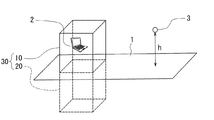•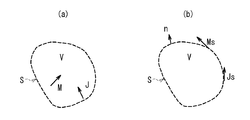•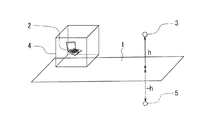•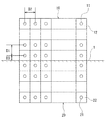•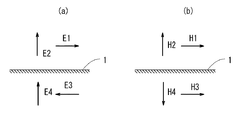•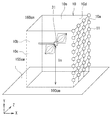•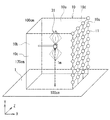•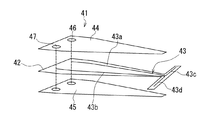•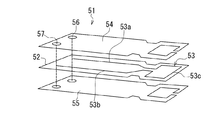•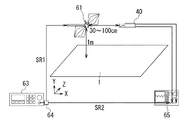•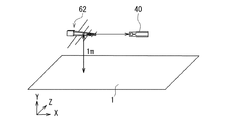•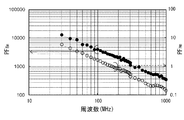••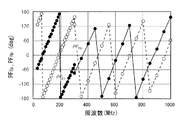•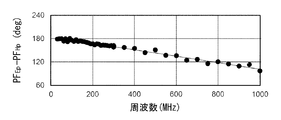•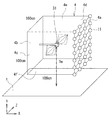•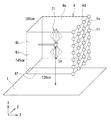•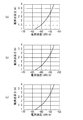•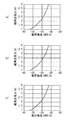•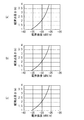•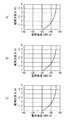••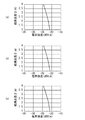•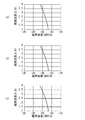••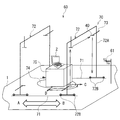•••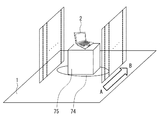•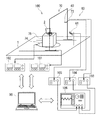••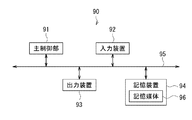•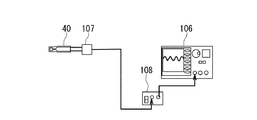•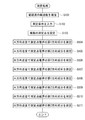••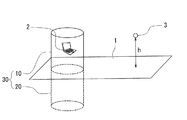•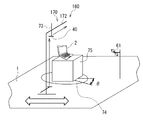•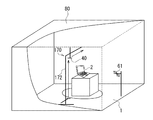•••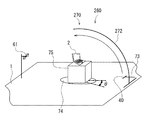•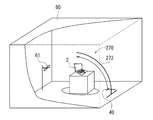•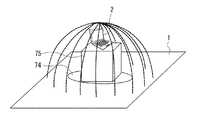•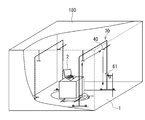••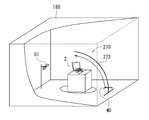## Classifications

• GPHYSICS
• G01MEASURING; TESTING
• G01RMEASURING ELECTRIC VARIABLES; MEASURING MAGNETIC VARIABLES
• G01R29/00Arrangements for measuring or indicating electric quantities not covered by groups G01R19/00 - G01R27/00
• G01R29/08Measuring electromagnetic field characteristics
• G01R29/0864Measuring electromagnetic field characteristics characterised by constructional or functional features
• G01R29/0871Complete apparatus or systems; circuits, e.g. receivers or amplifiers
• GPHYSICS
• G01MEASURING; TESTING
• G01RMEASURING ELECTRIC VARIABLES; MEASURING MAGNETIC VARIABLES
• G01R29/00Arrangements for measuring or indicating electric quantities not covered by groups G01R19/00 - G01R27/00
• G01R29/08Measuring electromagnetic field characteristics
• G01R29/0807Measuring electromagnetic field characteristics characterised by the application
• G01R29/0814Field measurements related to measuring influence on or from apparatus, components or humans, e.g. in ESD, EMI, EMC, EMP testing, measuring radiation leakage; detecting presence of micro- or radiowave emitters; dosimetry; testing shielding; measurements related to lightning
• G01R29/0821Field measurements related to measuring influence on or from apparatus, components or humans, e.g. in ESD, EMI, EMC, EMP testing, measuring radiation leakage; detecting presence of micro- or radiowave emitters; dosimetry; testing shielding; measurements related to lightning rooms and test sites therefor, e.g. anechoic chambers, open field sites or TEM cells
• GPHYSICS
• G01MEASURING; TESTING
• G01RMEASURING ELECTRIC VARIABLES; MEASURING MAGNETIC VARIABLES
• G01R29/00Arrangements for measuring or indicating electric quantities not covered by groups G01R19/00 - G01R27/00
• G01R29/08Measuring electromagnetic field characteristics
• G01R29/0864Measuring electromagnetic field characteristics characterised by constructional or functional features
• G01R29/0892Details related to signal analysis or treatment; presenting results, e.g. displays; measuring specific signal features other than field strength, e.g. polarisation, field modes, phase, envelope, maximum value

## Abstract

PROBLEM TO BE SOLVED: To easily and accurately estimate an electromagnetic field at a virtual observation point farther from a radiation source of an electromagnetic wave on the basis of an electromagnetic field measured in the vicinity of the radiation source under the condition that a ground plane exists.SOLUTION: In a method for estimating a far electromagnetic field, a measurement surface 10 is assumed which forms such a closed surface surrounding a radiation source 2 together with a ground plane 1 that a virtual observation point 3 is not included in a space formed on the inside of the closed surface, and a plurality of measurement points are set on the measurement surface 10, and electromagnetic fields at the plurality of measurement points are measured. Electromagnetic fields at a plurality of mirror image measurement points on a mirror image measurement surface 20 plane-symmetrical to the measurement surface 10 are calculated on the basis of the electromagnetic fields at the plurality of measurement points. Then, a far electromagnetic field at the observation point 3 is estimated on the basis of the electromagnetic fields at the plurality of measurement points and the plurality of mirror image measurement points.

## Description

The present invention measures an electromagnetic field from an electromagnetic radiation source such as an electronic device in the vicinity of the radiation source, and estimates an electromagnetic field at a virtual observation point farther from the radiation source based on the measured electromagnetic field. The present invention relates to a far electromagnetic field estimation method and apparatus, and a near electromagnetic field measurement apparatus suitable for implementing the far electromagnetic field estimation method.

In general, in a test for measuring radiated emission from electronic equipment, etc., radiation is mainly performed at a position 10 m or 3 m away from the emission source of the radiated emission due to internationally defined test conditions and test methods. Interference measurements are taken. A test for measuring a radiation disturbance wave in a frequency band from 30 MHz to 1 GHz is generally performed by placing an object to be measured as a radiation source on or above a ground plane (metal floor surface).

In general, a test for measuring radiated disturbances is performed in an anechoic chamber satisfying the suitability of an open site or an internationally defined site. The anechoic chamber is configured by attaching an electromagnetic wave absorber to the wall surface of the shield room. In this anechoic chamber, the size and shape of the shield room and the type of electromagnetic wave absorber are selected according to the purpose of use, the frequency of the electromagnetic wave used, and the like. The main anechoic chambers are a 10m anechoic chamber with a length of about 20m to 30m, a width of about 10m to 20m, a height of about 7m to 12m, a length of about 7m to 11m, and a width of about 4m to 7m. There is a 3m anechoic chamber with a height of about 5m to 7m. These are properly used according to the distance required for the test for measuring the radiated disturbance.

An anechoic chamber requires a relatively large length, width, and height as described above, the building facilities become large-scale, and the total investment and maintenance costs for the building facilities and various necessary equipments increase. have.

Therefore, there is a need for a small-scale device that can measure radiated interference in a smaller space. In response to this requirement, an apparatus for measuring a near-field electromagnetic field or a near-field electromagnetic field for a printed circuit board or the like, and a love equivalent theorem from the measured near-field electromagnetic field are used. A method for estimating a far electromagnetic field has been proposed.

For example, in Patent Document 1, a virtual rectangular parallelepiped or cylinder is set so as to surround the object to be measured, an electromagnetic field is measured so as to scan the surface of the rectangular parallelepiped or the cylinder, and based on the measured electromagnetic field. A method for estimating an electromagnetic field at a position farther from the object to be measured is described. Further, Patent Document 1 describes that only one of the other five surfaces may be scanned with one surface of a virtual rectangular parallelepiped as the ground surface.

In Non-Patent Document 1, a dipole antenna is selected as an object to be measured (radiation source), and the far electromagnetic field at the observation point is obtained as follows under the condition that the dipole antenna is arranged on the ground plane. A method is described. In this method, first, the amplitude and phase of the tangential component of the near electromagnetic field on the six surfaces set to surround the object to be measured are measured. Next, assuming that the ground plane is an infinite conductor flat plate, a mirror image observation point that is a mirror image of the observation point formed by the conductor flat plate is assumed, and the electromagnetic field is estimated at two points of the observation point and the mirror image observation point. Next, the estimated two electromagnetic fields are added in vector to obtain a far electromagnetic field at the observation point.

JP 2004-69372 A

Masataka Midori et al., "Fundamental study of 3m method estimation by near electromagnetic field measurement", IEICE technical report EMCJ2013-11 (2013-05)

In the method described in Patent Document 1, a virtual rectangular parallelepiped or cylinder is set so as to surround the object to be measured without considering the ground plane, and the rectangular parallelepiped or cylindrical surface is scanned, or the virtual plane is virtual. Only the other five surfaces are scanned with one surface of the rectangular parallelepiped as the ground surface. That is, this method does not consider the influence of the ground plane. For this reason, when this method is applied to a test performed under the condition that the object to be measured is placed on the ground plane as described above, the estimated electromagnetic field due to the influence of the ground plane becomes the actually measured electromagnetic field. It can be easily guessed that it is very different.

In the method described in Non-Patent Document 1, it is necessary to measure the near electromagnetic field on the lower surface of the object to be measured. However, in reality, since it is difficult to float the object to be measured in the air, it is difficult to measure the near electromagnetic field on the lower surface of the object to be measured. Therefore, it is difficult to apply this method to a test for measuring a radiated disturbance. In addition, this method requires a calculation process for estimating the electromagnetic field at the two observation points and the mirror image observation point, and a calculation process for adding the electromagnetic fields estimated at the two points in a vector manner. There is a problem that the calculation processing time is required.

The present invention has been made in view of such problems, and a first object of the present invention is from a radiation source based on an electromagnetic field measured in the vicinity of an electromagnetic wave radiation source under the condition that a ground plane exists. It is an object of the present invention to provide a far electromagnetic field estimation method and apparatus that can easily and accurately estimate an electromagnetic field at a virtual observation point at a farther position.

A second object of the present invention is to provide a near electromagnetic field measuring apparatus suitable for implementing the far electromagnetic field estimation method of the present invention.

The far electromagnetic field estimation method of the present invention is estimated to be formed at a virtual observation point away from a radiation source by an electromagnetic wave radiation source arranged in one of two spaces partitioned by a ground plane. This is a method for obtaining at least one of an electric field and a magnetic field.

The far electromagnetic field estimation method of the present invention is:
Assuming a measurement surface that forms a closed surface that is combined with the ground plane and surrounds the radiation source and does not include a virtual observation point in the space formed inside it, on the measurement surface A first procedure for setting a plurality of measurement points;
Measure at least one of a plurality of measurement point electric fields that are components in the tangential direction of the measurement surface of the electric field at a plurality of measurement points and a plurality of measurement point magnetic fields that are components in the tangential direction of the measurement surface of the magnetic field at the plurality of measurement points. A second procedure;
A plurality of mirror image measurement points having a plane-symmetric positional relationship with respect to the ground plane are set on the mirror image measurement surface having a plane-symmetric positional relationship with the measurement surface with the ground plane as a center, Based on at least one of the plurality of measurement point electric fields and the plurality of measurement point magnetic fields measured in the procedure, the plurality of mirror image measurement point electric fields and the plurality of mirror image measurement point electric fields that are tangential components of the mirror image measurement surface of the electric field at the plurality of mirror image measurement points A third procedure for calculating at least one of a plurality of mirror image measurement point magnetic fields which are components in a tangential direction of the mirror image measurement surface of the magnetic field at the mirror image measurement point;
At least one of the plurality of measurement point electric fields and the plurality of measurement point magnetic fields measured in the second procedure, and at least one of the plurality of mirror image measurement point electric fields and the plurality of mirror image measurement point magnetic fields calculated in the third procedure And a fourth procedure for calculating at least one of an electric field and a magnetic field estimated to be formed at a virtual observation point.

The far electromagnetic field estimation apparatus of the present invention is estimated to be formed at a virtual observation point away from a radiation source by an electromagnetic wave radiation source disposed in one of two spaces partitioned by a ground plane. It is an apparatus for obtaining at least one of an electric field and a magnetic field. The far electromagnetic field estimation device includes a near electromagnetic field measurement device and an arithmetic processing unit.

The near electromagnetic field measurement apparatus includes a probe for detecting at least one of an electric field and a magnetic field, a position control mechanism capable of changing a relative position of the probe with respect to a radiation source, and at least one of an electric field and a magnetic field using the probe and the position control mechanism. And a control unit for controlling the measurement.

The control unit
Assuming a measurement surface that forms a closed surface that is combined with the ground plane and surrounds the radiation source and does not include a virtual observation point in the space formed inside it, on the measurement surface A first operation for setting a plurality of measurement points;
Using the probe while controlling the position control mechanism, a plurality of measurement point electric fields that are components of the tangential direction of the measurement surface of the electric field at a plurality of measurement points and a tangential component of the measurement surface of the magnetic field at the plurality of measurement points And a second operation for measuring at least one of the plurality of measurement point magnetic fields.

The arithmetic processing unit
A plurality of mirror image measurement points having a plane-symmetric positional relationship with respect to the ground plane are set on the mirror image measurement surface having a plane-symmetric positional relationship with the measurement surface with the ground plane as a center, Based on at least one of the plurality of measurement point electric fields and the plurality of measurement point magnetic fields measured in operation, the plurality of mirror image measurement point electric fields and the plurality of mirror image measurement point electric fields that are tangential components of the mirror image measurement surface of the electric field at the plurality of mirror image measurement points A first calculation process for calculating at least one of a plurality of mirror image measurement point magnetic fields that are components in a tangential direction of the mirror image measurement surface of the magnetic field at the mirror image measurement point;
At least one of the plurality of measurement point electric fields and the plurality of measurement point magnetic fields measured in the second operation, and at least one of the plurality of mirror image measurement point electric fields and the plurality of mirror image measurement point magnetic fields calculated by the first calculation process Based on the above, a second calculation process for calculating at least one of an electric field and a magnetic field estimated to be formed at a virtual observation point is executed.

In the far electromagnetic field estimation method and apparatus of the present invention, the plurality of measurement points may be arranged so as to be aligned in two intersecting directions. In this case, for each of the two directions, the distance on the measurement surface between two adjacent measurement points may be ½ or less of the wavelength of the electromagnetic wave.

Further, in the far electromagnetic field estimation method and apparatus of the present invention, the plurality of measurement point electric fields, the plurality of measurement point magnetic fields, the plurality of mirror image measurement point electric fields, and the plurality of mirror image measurement point magnetic fields are each a horizontal component parallel to the ground plane. And a vertical component perpendicular to the ground plane. In this case, the horizontal components of the plurality of mirror image measurement point electric fields have the same amplitude as the horizontal components of the corresponding measurement point electric fields, but have the opposite phase. The vertical components of the plurality of mirror image measurement point electric fields have the same amplitude and phase as the corresponding vertical components of the measurement point electric fields. The horizontal components of the plurality of mirror image measurement point magnetic fields have the same amplitude and phase as the horizontal components of the corresponding measurement point magnetic fields. The vertical components of the plurality of mirror image measurement point magnetic fields have the same amplitude and opposite phases as the corresponding vertical components of the measurement point magnetic fields.

In the far electromagnetic field estimation method of the present invention, the radiation source may be arranged in an anechoic chamber having a metal floor surface constituting a ground plane. In the far electromagnetic field estimation apparatus of the present invention, the radiation source, the probe, and the position control mechanism may be arranged in an anechoic chamber having a metal floor surface that constitutes a ground plane.

In the far electromagnetic field estimation apparatus of the present invention, the probe may include an electric field detection unit that outputs a signal corresponding to the electric field and a magnetic field detection unit that outputs a signal corresponding to the magnetic field. The electric field detection unit includes an electric field antenna unit that generates a differential signal corresponding to the electric field, two electric field output ports that output the differential signal generated by the electric field antenna unit, and an electric field that shields a part of the electric field antenna unit. And a shield part. The magnetic field detection unit includes a magnetic field antenna unit that generates a differential signal corresponding to the magnetic field, two magnetic field output ports that output the differential signal generated by the magnetic field antenna unit, and a magnetic field that shields a part of the magnetic field antenna unit. And a shield part.

Further, the first correction information for correcting the amplitude and phase of the output signal of the electric field detection unit to values corresponding to the amplitude and phase of the electric field actually received by the electric field detection unit, and the output signal of the magnetic field detection unit Second correction information for correcting the amplitude and phase to values corresponding to the amplitude and phase of the magnetic field actually received by the magnetic field detector may be obtained in advance. In this case, in the second operation, only one of the plurality of measurement point electric fields and the plurality of measurement point magnetic fields may be measured. Then, the arithmetic processing unit includes the plurality of measurement point electric fields and the plurality of measurement point electric fields based on one of the plurality of measurement point electric fields and the plurality of measurement point magnetic fields measured in the second operation, and the first and second correction information. The other of the measurement point magnetic fields may be calculated. In this case, in the first calculation process, a plurality of mirror image measurement point electric fields and a plurality of mirror image measurement point magnetic fields may be calculated based on the plurality of measurement point electric fields and the plurality of measurement point magnetic fields. Further, in the second calculation process, it is estimated that a virtual observation point is formed based on a plurality of measurement point electric fields, a plurality of measurement point magnetic fields, a plurality of mirror image measurement point electric fields, and a plurality of mirror image measurement point magnetic fields. At least one of an electric field and a magnetic field may be calculated.

The near electromagnetic field measurement apparatus of the present invention measures electric fields at a plurality of measurement points on a measurement surface assumed to surround an electromagnetic wave radiation source disposed in one of two spaces partitioned by a ground plane. An apparatus for measuring at least one of a plurality of measurement point electric fields that are components in the tangential direction of the surface and a plurality of measurement point magnetic fields that are components in the tangential direction of the measurement surface of the magnetic field at the plurality of measurement points.

The near electromagnetic field measurement apparatus of the present invention includes a probe for detecting at least one of an electric field and a magnetic field, a position control mechanism capable of changing the relative position of the probe with respect to a radiation source, and an electric field and a magnetic field using the probe and the position control mechanism. A control unit that controls at least one of the measurements.

The control unit
The measurement surface is assumed to be a closed surface that surrounds the radiation source and that is combined with the ground plane and does not include a virtual observation point in the space formed inside it. A first operation for setting a plurality of measurement points on the top;
While controlling the position control mechanism, the probe is used to perform a second operation for measuring at least one of the plurality of measurement point electric fields and the plurality of measurement point magnetic fields.

In the near electromagnetic field measurement apparatus of the present invention, the plurality of measurement points may be arranged in two intersecting directions. In this case, for each of the two directions, the distance on the measurement surface between two adjacent measurement points may be ½ or less of the wavelength of the electromagnetic wave.

Moreover, in the near electromagnetic field measurement apparatus of the present invention, the radiation source, the probe, and the position control mechanism may be arranged in an anechoic chamber having a metal floor surface constituting a ground plane.

In the near electromagnetic field measurement apparatus of the present invention, the probe may include an electric field detection unit that outputs a signal corresponding to the electric field and a magnetic field detection unit that outputs a signal corresponding to the magnetic field. The electric field detection unit includes an electric field antenna unit that generates a differential signal corresponding to the electric field, two electric field output ports that output the differential signal generated by the electric field antenna unit, and an electric field that shields a part of the electric field antenna unit. And a shield part. The magnetic field detection unit includes a magnetic field antenna unit that generates a differential signal corresponding to the magnetic field, two magnetic field output ports that output the differential signal generated by the magnetic field antenna unit, and a magnetic field that shields a part of the magnetic field antenna unit. And a shield part.

In the far electromagnetic field estimation method and apparatus of the present invention, a plurality of measurement points are set on the measurement surface, at least one of the plurality of measurement point electric fields and the plurality of measurement point magnetic fields is measured, and a plurality of measurement point electric fields are measured on the mirror image measurement surface. A mirror image measurement point is set, and at least one of the plurality of mirror image measurement point electric fields and the plurality of mirror image measurement point magnetic fields is calculated based on at least one of the plurality of measurement point electric fields and the plurality of measurement point magnetic fields. Then, based on at least one of the measured plurality of measurement point electric fields and the plurality of measurement point magnetic fields, and at least one of the calculated plurality of mirror image measurement point electric fields and the plurality of mirror image measurement point magnetic fields, a virtual observation point is obtained. At least one of an electric field and a magnetic field estimated to be formed is calculated. Thus, according to the present invention, the electromagnetic field at a virtual observation point farther from the radiation source is calculated based on the electromagnetic field measured in the vicinity of the electromagnetic radiation source under the condition that the ground plane exists. Thus, it is possible to easily and accurately estimate.

Further, according to the near electromagnetic field measurement apparatus of the present invention, it is possible to measure at least one of a plurality of measurement point electric fields and a plurality of measurement point magnetic fields necessary for carrying out the far electromagnetic field estimation method of the present invention. The effect of becoming.

It is explanatory drawing for demonstrating the outline | summary of the far electromagnetic field estimation method which concerns on the 1st Embodiment of this invention. It is explanatory drawing for demonstrating Love's equivalence theorem. It is explanatory drawing for demonstrating the far electromagnetic field estimation method of a comparative example. It is explanatory drawing which shows the some measurement point and the some mirror image measurement point in the far electromagnetic field estimation method which concerns on the 1st Embodiment of this invention. It is explanatory drawing for demonstrating the calculation method of the mirror image measurement point electric field and the mirror image measurement point magnetic field in the far electromagnetic field estimation method which concerns on the 1st Embodiment of this invention. It is explanatory drawing for demonstrating the method of the experiment conducted regarding the far electromagnetic field estimation method which concerns on the 1st Embodiment of this invention. It is explanatory drawing for demonstrating the method of the experiment conducted regarding the far electromagnetic field estimation method which concerns on the 1st Embodiment of this invention. It is a perspective view which decomposes | disassembles and shows the electric field detection part of an electromagnetic field probe. It is a perspective view which decomposes | disassembles and shows the magnetic field detection part of an electromagnetic field probe. It is explanatory drawing for demonstrating the method of calculating | requiring an electric field probe coefficient and a magnetic field probe coefficient. It is explanatory drawing for demonstrating the method of calculating | requiring an electric field probe coefficient and a magnetic field probe coefficient. It is a characteristic view which shows an electric field amplitude coefficient and a magnetic field amplitude coefficient. It is a characteristic view which shows ratio of an electric field amplitude coefficient and a magnetic field amplitude coefficient. It is a characteristic view which shows an electric field phase coefficient and a magnetic field phase coefficient. It is a characteristic view which shows the difference of an electric field phase coefficient and a magnetic field phase coefficient. It is explanatory drawing for demonstrating the conditions of the comparative example in experiment. It is explanatory drawing for demonstrating the conditions of the comparative example in experiment. It is a characteristic view which shows the experimental result in case electromagnetic waves are horizontally polarized waves with a frequency of 30 MHz. It is a characteristic view which shows the experimental result in case electromagnetic waves are horizontally polarized waves with a frequency of 80 MHz. It is a characteristic view which shows the experimental result in case electromagnetic waves are horizontally polarized waves with a frequency of 100 MHz. It is a characteristic view which shows the experimental result in case electromagnetic waves are horizontally polarized waves with a frequency of 200 MHz. It is a characteristic view which shows the experimental result in case electromagnetic waves are vertically polarized waves with a frequency of 30 MHz. It is a characteristic view which shows the experimental result in case electromagnetic waves are vertically polarized waves with a frequency of 80 MHz. It is a characteristic view which shows the experimental result in case electromagnetic waves are vertically polarized waves with a frequency of 100 MHz. It is a characteristic view which shows the experimental result in case electromagnetic waves are vertically polarized waves with a frequency of 200 MHz. 1 is a perspective view showing a near electromagnetic field measuring apparatus according to a first embodiment of the present invention. It is a perspective view which shows an example of arrangement | positioning of the near electromagnetic field measuring apparatus shown in FIG. It is explanatory drawing for demonstrating operation | movement of the near electromagnetic field measuring apparatus shown in FIG. It is explanatory drawing for demonstrating operation | movement of the near electromagnetic field measuring apparatus shown in FIG. It is explanatory drawing which shows the structure of the far electromagnetic field estimation apparatus which concerns on the 1st Embodiment of this invention. It is a block diagram which shows the main components of the far electromagnetic field estimation apparatus which concerns on the 1st Embodiment of this invention. It is a block diagram which shows the structure of the computer in FIG. It is explanatory drawing which shows the modification of the far electromagnetic field estimation apparatus shown in FIG. It is a flowchart which shows the measurement process in the far electromagnetic field estimation method which concerns on the 1st Embodiment of this invention. It is a flowchart which shows the arithmetic processing in the far electromagnetic field estimation method which concerns on the 1st Embodiment of this invention. It is explanatory drawing for demonstrating the outline | summary of the far electromagnetic field estimation method which concerns on the 2nd Embodiment of this invention. It is a perspective view which shows the near electromagnetic field measuring apparatus which concerns on the 2nd Embodiment of this invention. It is a perspective view which shows an example of arrangement | positioning of the near electromagnetic field measuring apparatus shown in FIG. It is explanatory drawing for demonstrating arrangement | positioning of the several measurement point in the 2nd Embodiment of this invention. It is explanatory drawing for demonstrating the outline | summary of the far electromagnetic field estimation method which concerns on the 3rd Embodiment of this invention. It is a perspective view which shows the near electromagnetic field measuring device which concerns on the 3rd Embodiment of this invention. It is a perspective view which shows an example of arrangement | positioning of the near electromagnetic field measuring apparatus shown in FIG. It is explanatory drawing for demonstrating operation | movement of the near electromagnetic field measuring apparatus shown in FIG.

[First Embodiment]
Hereinafter, embodiments of the present invention will be described in detail with reference to the drawings. First, an overview of the far electromagnetic field estimation method according to the first embodiment of the present invention will be described with reference to FIG.

As shown in FIG. 1, in the far electromagnetic field estimation method according to the present embodiment, under the condition that the electromagnetic wave radiation source 2 is arranged in one of the two spaces partitioned by the ground plane 1, In this method, at least one of an electric field and a magnetic field estimated to be formed at a virtual observation point 3 away from the radiation source 2 is obtained. The far electromagnetic field estimation method according to the present embodiment is used, for example, in a test for measuring a radiation disturbance wave radiated from the radiation source 2 in accordance with the EMC standard.

The ground plane 1 is an equipotential surface, and is realized by, for example, a metal floor surface. As shown in FIG. 1, the two spaces defined by the ground plane 1 are the space above the ground plane 1 and the space below the ground plane 1 when the ground plane 1 is positioned horizontally. It is space. In FIG. 1, the radiation source 2 is disposed in a space above the ground plane 1. The radiation source 2 is, for example, an electronic device that generates electromagnetic waves. Such a situation is, for example, a situation in which the radiation source 2 is arranged in an anechoic chamber having a metal floor that constitutes the ground plane 1. In FIG. 1, the height of the observation point 3 with respect to the ground plane 1 is represented by the symbol h.

The far electromagnetic field estimation method according to the present embodiment includes the following first to fourth procedures. In the first procedure, a measurement is performed that forms a closed surface that surrounds the radiation source 2 together with the ground plane 1 and that does not include the virtual observation point 3 in the space formed inside thereof. A plurality of measurement points are set on the measurement surface 10 assuming the surface 10. The plurality of measurement points are positions where an electromagnetic field in the vicinity of the radiation source 2 is measured. In FIG. 1, a plurality of measurement points are not shown. The plurality of measurement points will be described in detail later.

In the present embodiment, the shape of the measurement surface 10 is a shape consisting of the remaining five surfaces excluding one of the six surfaces of the rectangular parallelepiped. The measurement surface 10 is arranged in such a posture that an opening at a position of one surface removed from the six surfaces of the rectangular parallelepiped is closed by the ground plane 1. Therefore, the measurement surface 10 is combined with the ground plane 1 to form six rectangular parallelepiped surfaces. In the present invention, the shape of the measurement surface 10 is not limited to the shape shown in FIG. Other examples of the shape of the measurement surface 10 are shown in other embodiments.

In the second procedure, a plurality of measurement point electric fields that are tangential components of the measurement surface 10 of the electric field at a plurality of measurement points and a plurality of measurements that are tangential components of the magnetic field measurement surface 10 at the plurality of measurement points. Measure at least one of the point magnetic fields.

In the third procedure, on the mirror image measurement surface 20 having a plane symmetry with respect to the measurement plane 10 with the ground plane 1 as the center, a plurality of measurement points having a plane symmetry with respect to the plurality of measurement points with the ground plane 1 as the center. Set the mirror image measurement point. In the example shown in FIG. 1, the mirror image measurement surface 20 has a shape consisting of the remaining five surfaces excluding one of the six surfaces of the rectangular parallelepiped, like the measurement surface 10. The mirror image measurement surface 20 is arranged on the side opposite to the measurement surface 10 with the ground plane 1 as the center, with an opening being closed by the ground plane 1. Therefore, the measurement surface 10 and the mirror image measurement surface 20 are joined together to form a closed surface 30 composed of six rectangular parallelepipeds. In FIG. 1, a plurality of mirror image measurement points are not shown. The plurality of mirror image measurement points will be described in detail later.

In the third procedure, a component in the tangential direction of the mirror image measurement surface of the electric field at the plurality of mirror image measurement points is further based on at least one of the plurality of measurement point electric fields and the plurality of measurement point magnetic fields measured in the second procedure. And at least one of a plurality of mirror image measurement point magnetic fields, which are components in the tangential direction of the mirror image measurement surface of the mirror image measurement point and the magnetic field at the plurality of mirror image measurement points.

In the fourth procedure, at least one of the plurality of measurement point electric fields and the plurality of measurement point magnetic fields measured in the second procedure, the plurality of mirror image measurement point electric fields and the plurality of mirror image measurement points calculated in the third procedure. Based on at least one of the magnetic fields, at least one of an electric field and a magnetic field estimated to be formed at the virtual observation point 3 is calculated. Hereinafter, at least one of an electric field and a magnetic field estimated to be formed at the virtual observation point 3 is also referred to as a far electromagnetic field at the virtual observation point 3.

In the fourth procedure, for example, the far electromagnetic field is calculated using Love's equivalence theorem. Here, the Love equivalence theorem will be described with reference to FIG. First, as shown in FIG. 2A, a region V including a radiation source is considered inside the closed surface S, and a current J and a magnetic current M due to the radiation source exist inside the region V, and the surface S It is assumed that an electric field E and a magnetic field H are generated above. J, M, E, and H are all vectors including time factors.

Next, as shown in FIG. 2B, the radiation source is removed from the inside of the region V, and an electric field E and a magnetic field H are generated on the surface S instead of the current J and the magnetic current M inside the region V. Such an equivalent surface current Js and surface magnetic current Ms are assumed on the surface S. Js and Ms are both vectors including a time factor. Js and Ms are represented by the following formulas (1) and (2) using E and H, respectively. Note that n is a unit normal vector of the surface S.

Js = n × H (1)
Ms = −n × E (2)

When the surface current Js and the surface magnetic current Ms are distributed on the surface S, the electric field E (r) and the magnetic field H (r) at the observation point at a distance r from the radiation source are expressed by the following equations (3 ) And (4).

E (r) = ∫ S [−jηk {JsG + (1 / k 2 ) · Js · ▽▽ G} −Ms × ▽ G] dS
... (3)
H (r) = ∫ S [−j · (1 / η) · k {MsG + (1 / k 2 ) · Ms · ▽▽ G} + Js × ▽ G] dS (4)

S in the expressions (3) and (4) represents the area along the surface S. Further, G, ▽ G, Js · ▽▽ G, Ms · ▽▽ G in the formulas (3) and (4) are as follows. Here, eta is intrinsic impedance, Green's function G is free space, k is 2 [pi / lambda, lambda is electromagnetic radiation wavelengths, dS is small area in the surface S, the r V is a unit direction vector pointing to the observation point.

G = exp (−jkr) / 4πr
G = {-(1 + jkr) / r} Gr V
(G / r) = {− (2 + jkr) / r 2 } Gr V
Js · ▽▽ G = (Js · r V ) {-▽ (G / r) -jk ▽ G}
= (Js · r V ) {− k 2 −2k 2 / jkr−2k 2 / (jkr) 2 } Gr V
Ms · ▽▽ G = (Ms · r V ) {-▽ (G / r) -jk ▽ G}
= (Ms · r V ) {− k 2 −2k 2 / jkr−2k 2 / (jkr) 2 } Gr V

In equations (3) and (4), if the distribution of components in the tangential direction of the surface S of the electromagnetic field on the surface S is correctly known, it can be used as a wave source to observe any position from the uniqueness of the electromagnetic field solution. This means that the electromagnetic field at a point can be determined. Since the equivalent surface current Js and surface magnetic current Ms on the surface S can be expressed by Fourier transform, in order to accurately obtain the electromagnetic field at the observation point at an arbitrary position, Fourier transform From the sampling theorem, which is a property, the interval between positions where the tangential component of the surface S of the electromagnetic field on the surface S is measured must be λ / 2 or less.

In the present embodiment, the closed surface 30 formed by combining the measurement surface 10 and the mirror image measurement surface 20 corresponds to the closed surface S in the above description. Further, the plurality of measurement point electric fields, the plurality of measurement point magnetic fields, the plurality of mirror image measurement point electric fields, and the plurality of mirror image measurement point magnetic fields correspond to components in the tangential direction of the surface S of the electromagnetic field on the surface S.

When calculating the far electromagnetic field at the virtual observation point 3 using the Love equivalence theorem, the fourth procedure uses the equations (1) and (2), and uses a plurality of measurement point electric fields and a plurality of measurement points. Based on the point magnetic field, the plurality of mirror image measurement point electric fields, and the plurality of mirror image measurement point magnetic fields, the distribution of the surface current Js and the surface magnetic current Ms on the surface 30 is obtained. In the fourth procedure, the far electromagnetic field at the virtual observation point 3 is further calculated from the distribution of the surface current Js and the surface magnetic current Ms on the surface 30 using the equations (3) and (4).

Next, the effects of the far electromagnetic field estimation method according to the present embodiment will be described in comparison with the far electromagnetic field estimation method of the comparative example. First, a far electromagnetic field estimation method of a comparative example will be described with reference to FIG. The far electromagnetic field estimation method of this comparative example is the method described in Non-Patent Document 1. As shown in FIG. 3, in the far electromagnetic field estimation method of the comparative example, first, the measurement surface 4 is assumed so as to surround the radiation source 2 arranged in the space above the ground plane 1. The measurement surface 4 is composed of, for example, six rectangular parallelepiped surfaces. Next, the amplitude and phase of the tangential component of the near electromagnetic field on the measurement surface 4 are measured. Next, a mirror image observation point 5 that is a mirror image of the observation point 3 formed by the ground plane 1 is assumed. As shown in FIG. 3, when the observation point 3 is at a height h with respect to the ground plane 1, the mirror image observation point 5 is located on the opposite side of the observation point 3 with respect to the ground plane 1. It is in the position of -h. Next, the electromagnetic field is estimated at two points, observation point 3 and mirror image observation point 5. Next, the estimated two electromagnetic fields are added in a vector manner to obtain the far electromagnetic field at the observation point 3.

The far electromagnetic field estimation method of the comparative example needs to measure the near electromagnetic field even on the surface located below the radiation source 2 (between the radiation source 2 and the ground plane 1) among the six surfaces constituting the measurement surface 4. There is. However, in reality, it is difficult to float the object to be measured as the radiation source 2 in the air, so it is difficult to measure the near electromagnetic field on the surface located below the radiation source 2. Therefore, it is difficult to apply this method to a test for measuring a radiated disturbance. In addition, this method requires a calculation process for estimating the electromagnetic field at two points of the observation point 3 and the mirror image observation point 5, and a calculation process for adding the electromagnetic fields estimated at the two points in a vector form. There is a problem that a lot of calculation processing time is required.

On the other hand, in the far electromagnetic field estimation method according to the present embodiment, it is not necessary to measure the electromagnetic field on a surface located below the radiation source 2 (between the radiation source 2 and the ground plane 1). Therefore, in the present embodiment, it is possible to easily measure the electromagnetic field in the vicinity of the radiation source 2 necessary for estimating the far electromagnetic field at the observation point 3.

In the present embodiment, the electromagnetic field is measured on the surface located below the radiation source 2, the surface current Js and the surface magnetic current Ms are calculated on the surface, and the surface current Js and the surface magnetic current Ms on the surface are calculated. The process of calculating the electromagnetic field at the observation point 3 and the mirror image observation point 5 is not required. Further, in the present embodiment, it is not necessary to perform a calculation process in which the electromagnetic field is estimated at two points, that is, the observation point 3 and the mirror image observation point 5, and the electromagnetic fields estimated at the two points are added in a vector form. It is only necessary to estimate the far electromagnetic field. From these things, according to this Embodiment, it is possible to shorten the time of a measurement and arithmetic processing compared with a comparative example.

In the present embodiment, since at least one of a plurality of mirror image measurement point electric fields and a plurality of mirror image measurement point magnetic fields is used in estimating the far electromagnetic field at the observation point 3, the influence of the ground plane 1 is affected. Has been taken into account.

From the above, according to the present embodiment, based on the electromagnetic field measured in the vicinity of the radiation source 2 under the condition that the ground plane 1 exists, a virtual observation at a position farther from the radiation source 2 is performed. The electromagnetic field at the point 3 can be estimated easily and accurately.

Next, with reference to FIG. 4, a plurality of measurement points and a plurality of mirror image measurement points in the present embodiment will be described. As shown in FIG. 4, in the present embodiment, the plurality of measurement points 11 are arranged on the measurement surface 10 so as to be arranged in two intersecting directions. More specifically, in the present embodiment, each of the five surfaces constituting the measurement surface 10 is divided into a plurality of regions 12 in a lattice shape. The plurality of measurement points 11 are respectively arranged at predetermined positions on the outer edge or inside of the plurality of regions 12. The predetermined position may be, for example, the center of the region 12 or one of the four corners of the outer edge of the region 12. The area of one region 12 corresponds to dS in equations (3) and (4).

In each of the five surfaces constituting the measurement surface 10, the plurality of measurement points 11 adjacent to the boundary (ridge line) with the other surface are preferably arranged at positions very close to the boundary.

The mirror image measurement surface 20 has a plurality of mirror image regions 22 having a plane-symmetrical positional relationship with the plurality of regions 12 around the ground plane 1 and a plane-symmetrical positional relationship with the plurality of measurement points 11 around the ground plane 1. A plurality of mirror image measurement points 21 are set.

Here, in one of the two directions in which the plurality of measurement points 11 are arranged, the distance on the measurement surface 10 between two adjacent measurement points 11 is D1, and the other of the two directions in which the plurality of measurement points 11 are arranged. The distance on the measurement surface 10 between two adjacent measurement points 11 is D2. D1 and D2 are set to a value greater than 0 and less than or equal to ½ of the wavelength of the electromagnetic wave according to the sampling theorem. D1 and D2 are preferably 1/6 or less of the wavelength of the electromagnetic wave.

A distance D3 between the measurement points 11 in the row 12 adjacent to the ground plane 1 and the ground plane 1 is set to a value greater than 0 and equal to or less than ¼ of the wavelength of the electromagnetic wave. This is because the distance between the measurement point 11 and the mirror image measurement point 21 that are adjacent to each other via the ground plane 1 is set to ½ or less of the wavelength of the electromagnetic wave.

When the wavelength of the electromagnetic wave to be measured has a range, D1, D2, and D3 can be determined based on the lower limit value of the wavelength of the electromagnetic wave. For example, when the upper limit value of the frequency of the electromagnetic wave is 1 GHz, the lower limit value of the wavelength of the electromagnetic wave is 30 cm. In this case, D1 and D2 are 15 cm or less, preferably 5 cm or less, and D3 is 7.5 cm or less.

The distance on the mirror image measurement surface 20 between two adjacent mirror image measurement points 21 and the distance between the mirror image measurement point 21 and the ground plane 1 in a row 22 adjacent to the ground plane 1 are both The same as in the case of the measurement point 11.

Next, a method for calculating the mirror image measurement point electric field and the mirror image measurement point magnetic field will be described with reference to FIG. First, a method for calculating a mirror image measurement point electric field will be described with reference to FIG. Here, it is assumed that each of the plurality of measurement point electric fields includes a horizontal component E1 parallel to the ground plane 1 and a vertical component E2 perpendicular to the ground plane 1. In this case, each of the plurality of mirror image measurement point electric fields also includes a horizontal component E3 parallel to the ground plane 1 and a vertical component E4 perpendicular to the ground plane 1. The horizontal component E3 and the vertical component E4 of the plurality of mirror image measurement point electric fields are given so as to satisfy the boundary condition of the ground plane 1 as follows. That is, the horizontal component E3 of the plurality of mirror image measurement point electric fields has the same amplitude as the horizontal component E1 of the corresponding measurement point electric field but has an opposite phase. The vertical component E4 of the plurality of mirror image measurement point electric fields has the same amplitude and phase as the corresponding vertical component E2 of the measurement point electric field. FIG. 5A shows a horizontal component E1 and a vertical component E2 of one measurement point electric field, and a horizontal component E3 and a vertical component E4 of one mirror image measurement point electric field corresponding thereto.

Next, a method for calculating the mirror image measurement point magnetic field will be described with reference to FIG. Here, it is assumed that each of the plurality of measurement point magnetic fields includes a horizontal component H1 parallel to the ground plane 1 and a vertical component H2 perpendicular to the ground plane 1. In this case, each of the plurality of mirror image measurement point magnetic fields also includes a horizontal component H3 parallel to the ground plane 1 and a vertical component H4 perpendicular to the ground plane 1. The horizontal component H3 and the vertical component H4 of the plurality of mirror image measurement point magnetic fields are given so as to satisfy the boundary condition of the ground plane 1 as follows. That is, the horizontal component H3 of the plurality of mirror image measurement point magnetic fields has the same amplitude and phase as the horizontal component H1 of the corresponding measurement point magnetic field. The vertical component H4 of the plurality of mirror image measurement point magnetic fields has the same amplitude but opposite phase to the corresponding vertical component H2 of the measurement point magnetic field. FIG. 5B shows a horizontal component H1 and a vertical component H2 of one measurement point magnetic field, and a horizontal component H3 and a vertical component H4 of one corresponding mirror image measurement point magnetic field.

[Verification experiment]
Hereinafter, with reference to FIGS. 6 to 25, an experiment conducted for verifying the validity of the far electromagnetic field estimation method according to the present embodiment will be described. In the experiment, the electromagnetic wave radiated from the radiation source 2 is horizontally polarized (hereinafter referred to as horizontal polarization), and the electromagnetic wave radiated from the radiation source 2 is vertically polarized (hereinafter referred to as vertical polarization). It was said to be the case of waves.)

FIG. 6 is an explanatory diagram for explaining an experimental method in the case of horizontal polarization. In the experiment, a biconical antenna 31 was used as the radiation source 2. In the case of horizontal polarization, as shown in FIG. 6, the biconical antenna 31 is disposed at a height of 1 m with respect to the ground plane 1 in a posture to radiate horizontally polarized electromagnetic waves.

Here, two directions parallel to and perpendicular to the ground plane 1 are defined as an X direction and a Z direction, and a direction perpendicular to the ground plane 1 is defined as a Y direction. In the case of horizontal polarization, a measurement surface 10 composed of five rectangular planes 10a, 10b, 10c, 10d, and 10e was assumed. The five planes correspond to five of the six planes of the rectangular parallelepiped. The planes 10 a and 10 b are perpendicular to the X direction, and each side is in contact with the ground plane 1. The planes 10 c and 10 d are perpendicular to the Z direction, and each side is in contact with the ground plane 1. The plane 10e is perpendicular to the Y direction and faces the ground plane 1. The dimensions in the X, Y, and Z directions of the rectangular parallelepiped formed by combining the measurement surface 10 and the ground plane 1 are 100 cm, 150 cm, and 160 cm, respectively. The distances from the biconical antenna 31 to the planes 10a and 10b are equal to each other, and the distances from the biconical antenna 31 to the planes 10c and 10d are also equal to each other. A plurality of measurement points 11 are arranged on the measurement surface 10. In each of the X, Y, and Z directions, the distance on the measurement surface 10 between two adjacent measurement points 11 is 10 cm.

FIG. 7 is an explanatory diagram for explaining an experimental method in the case of vertical polarization. In this case, as shown in FIG. 7, the biconical antenna 31 is disposed at a height of 1 m with respect to the ground plane 1 in a posture to radiate vertically polarized electromagnetic waves. Similarly to the case of horizontal polarization, a measurement surface 10 including five rectangular planes 10a, 10b, 10c, 10d, and 10e was assumed. The dimensions in the X, Y, and Z directions of the rectangular parallelepiped formed by combining the measurement surface 10 and the ground plane 1 are 100 cm, 170 cm, and 100 cm, respectively. The distances from the biconical antenna 31 to the planes 10a and 10b are equal to each other, and the distances from the biconical antenna 31 to the planes 10c and 10d are also equal to each other. A plurality of measurement points 11 are arranged on the measurement surface 10. In each of the X, Y, and Z directions, the distance on the measurement surface 10 between two adjacent measurement points 11 is 10 cm.

In the experiment, the measurement point electric field and the measurement point magnetic field at a plurality of measurement points 11 were measured using an electromagnetic field probe described later in the case of horizontal polarization and vertical polarization, respectively. There were four types of electromagnetic wave frequencies of 30 MHz, 80 MHz, 100 MHz, and 200 MHz. The measurement point electric field and the measurement point magnetic field in the case of horizontal polarization respectively correspond to the horizontal component of the measurement point electric field and the horizontal component of the measurement point magnetic field described with reference to FIG. The measurement point electric field and the measurement point magnetic field in the case of vertical polarization respectively correspond to the vertical component of the measurement point electric field and the vertical component of the measurement point magnetic field described with reference to FIG.

Here, the electromagnetic field probe will be described with reference to FIGS. 8 and 9. The electromagnetic field probe used in the experiment has an electric field detection unit 41 that outputs a signal according to an electric field, and a magnetic field detection unit 51 that outputs a signal according to a magnetic field. FIG. 8 is an exploded perspective view showing the electric field detector 41. FIG. 9 is an exploded perspective view showing the magnetic field detection unit 51. The electromagnetic field probe is configured by combining an electric field detection unit 41 and a magnetic field detection unit 51. Hereinafter, the electromagnetic field probe is represented by reference numeral 40.

As shown in FIG. 8, the electric field detection unit 41 includes a printed circuit board 42 including an electric field antenna unit 43 formed of a patterned conductor layer, and shield plates 44 and 45 formed of conductors disposed above and below the printed circuit board 42. And. The electric field antenna unit 43 constitutes a dipole antenna. The electric field antenna portion 43 includes two cable portions 43a and 43b, an element 43c connected to one end of the cable portion 43a, and an element 43d connected to one end of the cable portion 43b. Each of the two elements 43c and 43d has a length of 10 mm.

The electric field detection unit 41 further includes two electric field output ports 46 and 47 including two through holes penetrating the shield plates 44 and 45 and the printed board 42. The electric field output port 46 is connected to the other end of the cable portion 43a, and the electric field output port 47 is connected to the other end of the cable portion 43b. The shield plates 44 and 45 have a shape in which the cable portions 43 a and 43 b that are part of the electric field antenna portion 43 are sandwiched from above and below. The electric field antenna 43 and the electric field output ports 46 and 47 are insulated from the shield plates 44 and 45. The shield plates 44 and 45 are electrically connected to each other through a plurality of through holes (not shown). The shield plates 44 and 45 constitute an electric field shield part that shields a part of the electric field antenna part 43. The electric field antenna unit 43 generates a differential signal corresponding to the detected electric field. The two electric field output ports 46 and 47 output the differential signals generated by the electric field antenna unit 43. The differential signal is composed of two signals having the same amplitude and opposite phases.

As shown in FIG. 9, the magnetic field detection unit 51 includes a printed circuit board 52 including a magnetic field antenna unit 53 made of a patterned conductor layer, and shield plates 54 and 55 made of conductors arranged above and below the printed circuit board 52. And. The magnetic field antenna unit 53 constitutes a loop antenna. The magnetic field antenna portion 53 includes two cable portions 53a and 53b, and a loop portion 53c that connects one end of the cable portion 53a and one end of the cable portion 53b. The shape of the loop portion 53c is a substantially square having a side length of 20 mm.

The magnetic field detection unit 51 further includes two magnetic field output ports 56 and 57 each including two through holes penetrating the shield plates 54 and 55 and the printed circuit board 52. The magnetic field output port 56 is connected to the other end of the cable portion 53a, and the magnetic field output port 57 is connected to the other end of the cable portion 53b. The shield plates 54 and 55 have a shape that sandwiches a part of the magnetic field antenna portion 53. Specifically, the shield plates 54 and 55 have a shape that sandwiches the entire cable portions 53a and 53b and sandwiches a portion excluding a part of the loop portion 53c. The magnetic field antenna unit 53 and the magnetic field output ports 56 and 57 are insulated from the shield plates 54 and 55. The shield plates 54 and 55 are electrically connected to each other through a plurality of through holes (not shown). The shield plates 54 and 55 constitute a magnetic field shield part that shields a part of the magnetic field antenna part 53. The magnetic field antenna unit 53 generates a differential signal corresponding to the detected magnetic field. The two magnetic field output ports 56 and 57 output the differential signal generated by the magnetic field antenna unit 53.

As described above, the electric field detection unit 41 and the magnetic field detection unit 51 both generate a differential signal. Such an electric field detection unit 41 and a magnetic field detection unit 51 have a feature that they can cancel out stationary noise generated in the same phase between two signals constituting a differential signal, and are less dependent on the detection direction.

In order to measure the amplitude and phase of the electric field and the amplitude and phase of the magnetic field using the electromagnetic field probe 40, the amplitude and phase of the output signal of the electric field detection unit 41 are set according to the inherent reception characteristics of the electric field detection unit 41. The output of the magnetic field detection unit 51 in accordance with the first correction information for correcting the value to the value corresponding to the amplitude and phase of the electric field actually received by the electric field detection unit 41 and the unique reception characteristics of the magnetic field detection unit 51 The second correction information for correcting the amplitude and phase of the signal to values corresponding to the amplitude and phase of the magnetic field actually received by the magnetic field detector 51 is necessary. Hereinafter, the first correction information is referred to as an electric field probe coefficient, and the second correction information is referred to as a magnetic field probe coefficient. In the experiment, the electric field probe coefficient and the magnetic field probe coefficient were obtained in advance by the method described below.

10 and 11 are explanatory diagrams for explaining a method for obtaining an electric field probe coefficient and a magnetic field probe coefficient (hereinafter referred to as a method for obtaining a probe coefficient). In the method of obtaining the probe coefficient, first, a reference electromagnetic wave is emitted from the radiation source, the electromagnetic wave probe 40 detects the electromagnetic wave, and basic data for obtaining the electric field probe coefficient and the magnetic field probe coefficient is collected. Here, the biconical antenna 61 shown in FIG. 10 and the log periodic antenna 62 shown in FIG. 11 were used as radiation sources.

First, the case where the biconical antenna 61 is used as a radiation source will be described with reference to FIG. In the method of obtaining the probe coefficient, in addition to the electromagnetic field probe 40 and the biconical antenna 61, a reference signal generator 63, a signal distributor 64, and a receiver 65 are used. The reference signal generator 63 generates a reference signal. The reference signal is divided into two reference signals SR1 and SR2 by the signal distributor 64. The reference signal SR1 is supplied to the biconical antenna 61 with a magnitude of 1V. The reference signal SR2 is supplied to the receiver 65. The biconical antenna 61 radiates electromagnetic waves based on the reference signal SR1. The electromagnetic field probe 40 detects this electromagnetic wave.

In the method of obtaining the probe coefficient, the biconical antenna 61 and the electromagnetic field probe 40 are arranged at a height of 1 m with respect to the ground plane 1. The distance between the biconical antenna 61 and the electromagnetic field probe 40 was changed at intervals of 10 cm in a range of 30 cm to 100 m. The biconical antenna 61 was arranged in a posture to radiate horizontally polarized electromagnetic waves. The electromagnetic field probe 40 is arranged in such an attitude that the electric field detection unit 41 detects the horizontal component of the electric field and the magnetic field detection unit 51 detects the vertical component of the magnetic field. The frequency of the electromagnetic wave radiated by the biconical antenna 61 was changed within the range of 30 MHz to 300 MHz. Two differential signals output from the electric field detection unit 41 and the magnetic field detection unit 51 of the electromagnetic field probe 40 are supplied to the receiver 65. The receiver 65 generates a plurality of measurement data corresponding to the received reference signal SR2 and the two differential signals. The plurality of measurement data is sent from the receiver 65 to a computer (not shown).

Next, a case where the log periodic antenna 62 is used as a radiation source will be described with reference to FIG. In this case, instead of the biconical antenna 61, a log periodic antenna 62 is disposed at a height of 1 m with respect to the ground plane 1. The distance between the log periodic antenna 62 and the electromagnetic field probe 40 was changed at 10 cm intervals in the range of 30 cm to 100 m. The log periodic antenna 62 was arranged in a posture to radiate horizontally polarized electromagnetic waves. The frequency of the electromagnetic wave radiated by the log periodic antenna 62 was changed within a range of 300 MHz to 1 GHz. Other conditions when the log periodic antenna 62 is used are the same as those when the biconical antenna 61 is used.

In the method of obtaining the probe coefficient, the electric field probe coefficient and the magnetic field probe coefficient were obtained as follows using the computer. First, the difference between the two signals constituting the differential signal output from the electric field detection unit 41 is obtained as the electric field detection signal SE, and the difference between the two signals constituting the differential signal output from the magnetic field detection unit 51 is determined as the magnetic field. The detection signal SH was obtained. Next, the value SE / SR2 obtained by dividing the electric field detection signal SE by the reference signal SR2 is obtained as the electric field measurement value Emeas, and the value SH / SR2 obtained by dividing the magnetic field detection signal SH by the reference signal SR2 is measured by the magnetic field. Determined as the value Hmeas. The electric field measurement value Emeas and the magnetic field measurement value Hmeas are basic data for obtaining the electric field probe coefficient and the magnetic field probe coefficient.

In the method of obtaining the probe coefficient, the measurement system shown in FIG. 10 and the measurement system shown in FIG. 11 are modeled, and the electric field calculation value Ecal, which is a calculation value corresponding to the electric field measurement value Emeas, is calculated by the moment method. The magnetic field calculation value Hcal, which is a calculation value corresponding to the magnetic field measurement value Hmeas, was obtained. Then, the electric field probe coefficient PF E and the magnetic field probe coefficient PF H were obtained by the following formulas (5) and (6).

PF E = Ecal / Emeas (5)
PF H = Hcal / Hmeas (6)

The electric field probe coefficient PF E and the magnetic field probe coefficient PF H have amplitude and phase information, respectively. Here, it referred to the amplitude information of which has an electric field probe coefficient PF E the electric field amplitude coefficient, called the phase information included in the electric field probe coefficient PF E the electric field phase factor, the magnetic field amplitude amplitude information included in the magnetic field probe factor PF H The phase information of the magnetic field probe coefficient PF H is called a magnetic field phase coefficient.

The above-mentioned electric field amplitude coefficient, electric field phase coefficient, magnetic field amplitude coefficient, and magnetic field phase coefficient are obtained by changing the distance between the antenna 61 or 62 and the electromagnetic field probe 40 and the frequency of the electromagnetic wave emitted by the antenna 61 or 62. It has been found that these coefficients for each frequency are substantially constant regardless of the distance between the antenna 61 or 62 and the electromagnetic field probe 40.

Here, the average values of the electric field amplitude coefficient, electric field phase coefficient, magnetic field amplitude coefficient, and magnetic field phase coefficient for each frequency (a plurality of coefficients obtained under different conditions of the distance between the antenna 61 or 62 and the electromagnetic field probe 40). Is an electric field amplitude coefficient PF Em , an electric field phase coefficient PF Ep , a magnetic field amplitude coefficient PF Hm , and a magnetic field phase coefficient PF Hp . In the method of obtaining the probe coefficient, the electric field amplitude coefficient PF Em is obtained as information on the amplitude of the electric field probe coefficient, the electric field phase coefficient PF Ep is obtained as information on the phase of the electric field probe coefficient, and the magnetic field amplitude coefficient is obtained as information on the amplitude of the magnetic field probe coefficient. PF Hm was obtained, and magnetic field phase coefficient PF Hp was obtained as information on the phase of the magnetic field probe coefficient. In the following description, the electric field probe coefficient PF E has an amplitude represented by an electric field amplitude coefficient PF Em and a phase represented by an electric field phase coefficient PF Ep , and the magnetic field probe coefficient PF H is a magnetic field amplitude coefficient. It is assumed that it has a phase represented by an amplitude represented by PF Hm and a magnetic field phase coefficient PF Hp .

FIG. 12 is a characteristic diagram showing the electric field amplitude coefficient PF Em and the magnetic field amplitude coefficient PF Hm . In FIG. 12, the horizontal axis represents frequency, and the vertical axis represents PF Em and PF Hm . In FIG. 12, a plurality of black circles represents the electric field amplitude coefficient PF Em for each frequency, and a plurality of white circles represents the magnetic field amplitude coefficient PF Hm for each frequency.

FIG. 13 is a characteristic diagram showing a ratio PF Em / PF Hm between the electric field amplitude coefficient PF Em and the magnetic field amplitude coefficient PF Hm . In FIG. 13, the horizontal axis represents frequency, and the vertical axis represents PF Em / PF Hm .

FIG. 14 is a characteristic diagram showing the electric field phase coefficient PF Ep and the magnetic field phase coefficient PF Hp . In FIG. 14, the horizontal axis indicates the frequency, and the vertical axis indicates PF Ep and PF Hp . The unit of PF Ep and PF Hp is degrees. In FIG. 14, a plurality of black circles represents the electric field phase coefficient PF Ep for each frequency, and a plurality of white circles represents the magnetic field phase coefficient PF Hp for each frequency.

FIG. 15 is a characteristic diagram showing a difference PF Ep −PF Hp between the electric field phase coefficient PF Ep and the magnetic field phase coefficient PF Hp . In FIG. 15, the horizontal axis indicates the frequency, and the vertical axis indicates PF Ep −PF Hp . The unit of PF Ep -PF Hp is degrees.

If the electric field probe coefficient PF E is obtained in advance with respect to the electromagnetic field probe 40 to be used, the amplitude and phase of the output signal of the electric field detector 41 are determined using the electric field probe coefficient PF E. Can be corrected to a value corresponding to the amplitude and phase of the electric field actually received by the electric field detector 41. Similarly, if the magnetic field probe coefficient PF H is obtained in advance for the electromagnetic field probe 40 to be used, the magnetic field probe coefficient PF H is used to change the amplitude and phase of the output signal of the magnetic field detection unit 51 to the magnetic field detection unit. The value can be corrected to a value corresponding to the amplitude and phase of the magnetic field actually received by the magnetic field detection unit 51. Specifically, the electric field after the correction is obtained by multiplying the electric field probe factor PF E to the output signal of the electric field detector 41, the magnetic field after the correction, the magnetic field probe coefficient to the output signal of the magnetic field detector 51 PF H Is obtained by multiplying

Further, regarding the electromagnetic field probe 40 to be used, the ratio PF Em / PF Hm of the electric field amplitude coefficient PF Em and the magnetic field amplitude coefficient PF Hm as shown in FIG. 13 and the electric field phase coefficient PF as shown in FIG. If the difference PF Ep −PF Hp between Ep and the magnetic field phase coefficient PF Hp is obtained, the amplitude and phase are measured for one of the electric field and the magnetic field, and the measurement result and the above ratio PF Em / PF Hm and the difference PF Ep Using −PF Hp , the amplitude and phase for the other of the electric and magnetic fields can be determined by calculation as follows.

When the amplitude and phase of the horizontal component of the electric field and the amplitude and phase of the vertical component of the electric field are measured, the amplitude of the vertical component of the magnetic field is obtained by dividing the amplitude of the horizontal component of the electric field by the ratio PF Em / PF Hm Can do. Further, the amplitude of the horizontal component of the magnetic field can be obtained by dividing the amplitude of the vertical component of the electric field by the ratio PF Em / PF Hm . Further, the phase of the vertical component of the magnetic field can be obtained by subtracting the difference PF Ep −PF Hp from the phase of the horizontal component of the electric field. Further, the phase of the horizontal component of the magnetic field can be obtained by subtracting the difference PF Ep −PF Hp from the phase of the vertical component of the electric field.

When the amplitude and phase of the horizontal component of the magnetic field and the amplitude and phase of the vertical component of the magnetic field are measured, the amplitude of the vertical component of the electric field is obtained by multiplying the amplitude of the horizontal component of the magnetic field by the ratio PF Em / PF Hm Can do. Also, the amplitude of the horizontal component of the electric field can be obtained by multiplying the amplitude of the vertical component of the magnetic field by the ratio PF Em / PF Hm . Further, the phase of the vertical component of the electric field can be obtained by adding the difference PF Ep −PF Hp to the phase of the horizontal component of the magnetic field. Further, the phase of the horizontal component of the electric field can be obtained by adding the difference PF Ep -PF Hp to the phase of the vertical component of the magnetic field.

In the experiment, for each of the measurement point electric field and the measurement point magnetic field at the plurality of measurement points 11 using the electromagnetic field probe 40 in the case of the horizontal polarization shown in FIG. 6 and the case of the vertical polarization shown in FIG. Amplitude and phase were measured. The amplitude and phase of each of the measurement point electric field and the measurement point magnetic field are values after correction using the electric field probe coefficient PF E and the magnetic field probe coefficient PF H.

In the experiment, next, a plurality of mirror image measurement points 21 (see FIG. 4) were set on the mirror image measurement surface 20 having a plane-symmetrical positional relationship with the measurement surface 10 with the ground plane 1 as the center. Based on the amplitude and phase of the measurement point electric field and the measurement point magnetic field at the plurality of measurement points 11, the amplitude and phase of the mirror image measurement point electric field and the mirror image measurement point magnetic field at the plurality of mirror image measurement points 21 were calculated. . Next, based on the respective amplitudes and phases of the measurement point electric field and the measurement point magnetic field at the plurality of measurement points 11, and the respective amplitudes and phases of the mirror image measurement point electric field and the mirror image measurement point magnetic field at the plurality of mirror image measurement points 21, Using expressions (1) and (2), the distribution of the surface current Js and the surface magnetic current Ms on the surface 30 (see FIG. 1) was obtained. Next, the far electromagnetic field at the virtual observation point 3 was obtained from the distribution of the surface current Js and the surface magnetic current Ms on the surface 30 using the equations (3) and (4). In the experiment, in particular, the electric field strength at the virtual observation point 3 was obtained. The virtual observation point 3 was assumed at a position 10 m away from the radiation source 2 (the biconical antenna 31) in the X direction. The height of the virtual observation point 3 with respect to the ground plane 1 (hereinafter referred to as observation point height) was changed within a range of 1 m to 4 m. Hereinafter, the electric field strength at the observation point 3 obtained in this way is referred to as electric field strength according to the present embodiment.

In the experiment, the theoretical value of the electric field strength at the observation point 3 was obtained using the moment method. In the experiment, the electric field strength at the observation point 3 was obtained using the far electromagnetic field estimation method of the comparative example shown in FIG. Here, with reference to FIG. 16 and FIG. 17, the conditions when the electric field strength at the observation point 3 is obtained using the far electromagnetic field estimation method of the comparative example will be described. Similar to the method of the experiment shown in FIGS. 6 and 7, in the comparative example, the measurement was performed separately for the case of horizontal polarization and for the case of vertical polarization.

FIG. 16 is an explanatory diagram for explaining the conditions of the comparative example in the case of horizontal polarization. In this case, the position and orientation of the biconical antenna 31 as the radiation source 2 are the same as those in the experiment shown in FIG. In the comparative example, as shown in FIG. 16, the measurement surface 4 including six rectangular planes 4 a, 4 b, 4 c, 4 d, 4 e, and 4 f is assumed so as to surround the biconical antenna 31. The six planes correspond to the six planes of the rectangular parallelepiped. The dimensions of the rectangular parallelepiped in the X, Y, and Z directions are 100 cm, 100 cm, and 160 cm, respectively. A plurality of measurement points 11 are arranged on the measurement surface 4. In each of X, Y, and Z directions, the distance on the measurement surface 4 between two adjacent measurement points 11 is 10 cm.

FIG. 17 is an explanatory diagram for explaining the conditions of the comparative example in the case of vertical polarization. The position and posture of the biconical antenna 31 as the radiation source 2 in this case are the same as in the experiment shown in FIG. In the comparative example, as shown in FIG. 17, a measurement surface 4 including six rectangular planes 4 a, 4 b, 4 c, 4 d, 4 e, and 4 f is assumed so as to surround the biconical antenna 31. The six planes correspond to the six planes of the rectangular parallelepiped. The dimensions of the rectangular parallelepiped in the X, Y, and Z directions are 100 cm, 140 cm, and 100 cm, respectively. A plurality of measurement points 11 are arranged on the measurement surface 4. In each of X, Y, and Z directions, the distance on the measurement surface 4 between two adjacent measurement points 11 is 10 cm.

In the comparative example, in the case of the horizontal polarization shown in FIG. 16 and the case of the vertical polarization shown in FIG. The amplitude and phase were measured. In the comparative example, next, assuming the mirror image observation point 5, the electric field was estimated at two points, the observation point 3 and the mirror image observation point 5. Next, the estimated two electric fields were added in vector, and the electric field strength at the observation point 3 was obtained. Hereinafter, the electric field strength at the observation point 3 obtained in this way is referred to as the electric field strength according to the comparative example.

The results of the experiment are shown in FIGS. FIG. 18 shows the experimental results when the electromagnetic wave is horizontally polarized with a frequency of 30 MHz. FIG. 19 shows the experimental results when the electromagnetic wave is horizontally polarized with a frequency of 80 MHz. FIG. 20 shows the experimental results when the electromagnetic wave is horizontally polarized with a frequency of 100 MHz. FIG. 21 shows experimental results when the electromagnetic waves are horizontally polarized with a frequency of 200 MHz. FIG. 22 shows experimental results when the electromagnetic waves are vertically polarized with a frequency of 30 MHz. FIG. 23 shows the experimental results when the electromagnetic waves are vertically polarized with a frequency of 80 MHz. FIG. 24 shows experimental results when the electromagnetic waves are vertically polarized with a frequency of 100 MHz. FIG. 25 shows experimental results when the electromagnetic waves are vertically polarized with a frequency of 200 MHz.

18 to 25, (a) shows the relationship between the observation point height and the theoretical value of the electric field strength. (B) has shown the relationship between observation point height and the electric field strength by this Embodiment. (C) has shown the relationship between observation point height and the electric field strength by a comparative example. In (a) to (c), the vertical axis represents the observation point height (m), and the horizontal axis represents the electric field strength (dBV / m).

As shown in FIGS. 18 to 25, the electric field strength according to the present embodiment substantially coincides with the theoretical value of the electric field strength, and also substantially coincides with the electric field strength according to the comparative example. From this result, it was confirmed that the far electromagnetic field estimation method according to the present embodiment is appropriate.

[Distant electromagnetic field estimation device and near electromagnetic field measurement device]
Hereinafter, the far electromagnetic field estimation apparatus and the near electromagnetic field measurement apparatus according to the present embodiment will be described with reference to FIGS. The far electromagnetic field estimation apparatus according to the present embodiment is an apparatus that implements the far electromagnetic field estimation method according to the present embodiment. That is, when the far electromagnetic field estimation device is formed at a virtual observation point 3 away from the radiation source 2 by the electromagnetic wave radiation source 2 arranged in one of the two spaces partitioned by the ground plane 1. At least one of an estimated electric field and magnetic field is obtained. The far electromagnetic field estimation apparatus includes a near electromagnetic field measurement apparatus according to the present embodiment and an arithmetic processing unit.

First, the near electromagnetic field measurement apparatus according to the present embodiment will be described with reference to FIG. The near electromagnetic field measuring apparatus 60 includes one or more probes that detect at least one of an electric field and a magnetic field, a position control mechanism 70 that can change the relative position of the probe with respect to the radiation source 2, and a probe and the position control mechanism 70. A control unit that controls measurement of at least one of the electric field and the magnetic field used. The probe in the present embodiment is particularly the electromagnetic field probe 40 having the electric field detection unit 41 shown in FIG. 8 and the magnetic field detection unit 51 shown in FIG. FIG. 26 does not show the control unit.

The control unit performs the first operation and the second operation. In the first operation, measurement is performed to form a closed surface that is combined with the ground plane 1 and surrounds the radiation source 2 and does not include the virtual observation point 3 in the space formed inside thereof. Assuming the surface 10 (see FIG. 1), a plurality of measurement points 11 (see FIG. 4) are set on the measurement surface 10. In the second operation, using the electromagnetic field probe 40 while controlling the position control mechanism 70, a plurality of measurement point electric fields that are components in the tangential direction of the measurement surface 10 of the electric field at the plurality of measurement points 11, and a plurality of measurement point electric fields At least one of a plurality of measurement point magnetic fields which are components in the tangential direction of the measurement surface 10 of the magnetic field at the measurement point 11 is measured. In the present embodiment, in particular, in the first operation, as shown in FIG. 1, a measurement surface 10 having a shape consisting of the remaining five surfaces excluding one of the six surfaces of the rectangular parallelepiped is assumed.

The position control mechanism 70 includes two rails 71 and 71 provided on the ground plane 1 and a movable frame 72. The movable frame 72 includes a frame main body 72A and four wheels 72B attached to the frame main body 72A and movable along the two rails 71 and 71. The frame main body 72A has two vertical portions that extend in the vertical direction above the two rails 71 and 71, and a horizontal portion that extends in the horizontal direction and connects the upper ends of the two vertical portions.

The position control mechanism 70 further includes an arm 73 that supports the electromagnetic field probe 40 and is attached to the frame body 72A so as to be movable along the frame body 72A. The arm 73 can change the posture of the electromagnetic field probe 40 so that the direction of the electric field and magnetic field detected by the electromagnetic field probe 40 can be changed.

The position control mechanism 70 further includes a disk-shaped turntable 74 attached to the ground plane 1. The turntable 74 is rotatable about an axis perpendicular to the ground plane 1. Here, a direction from the rotation center of the turntable 74 toward a predetermined point on the outer periphery of the turntable 74 is defined as a front direction of the turntable 74. The radiation source 2 is mounted, for example, on a gantry 75 disposed on the turntable 74.

Although not shown, the position control mechanism 70 further includes a driving device that moves the movable frame 72 along the two rails 71, 71, a driving device that moves the arm 73 along the frame main body 72A, and the arm 73. A drive device that changes the posture of the electromagnetic field probe 40 to be supported and a drive device that rotates the turntable 74 are provided. The control unit controls the plurality of driving devices and performs the second operation described above. A plurality of sets of the electromagnetic field probe 40 and the arm 73 may be provided.

The far electromagnetic field estimation apparatus according to the present embodiment further includes a reference point antenna 61. The reference point antenna 61 is disposed above the ground plane 1 and at a predetermined position outside the space surrounded by the measurement surface 10. The function of the reference point antenna 61 will be described in detail later.

FIG. 27 is a perspective view showing an example of the arrangement of the near electromagnetic field measurement apparatus 60 shown in FIG. In this example, the radiation source 2, the electromagnetic field probe 40, the position control mechanism 70, and the reference point antenna 61 are disposed in an anechoic chamber 80 having a metal floor surface constituting the ground plane 1. The anechoic chamber 80 is configured by attaching an electromagnetic wave absorber to the wall surface of the shield room excluding the metal floor surface.

Next, referring to FIG. 28 and FIG. 29, the near electromagnetic field measurement apparatus 60 when measuring at least one of the measurement point electric field and the measurement point magnetic field at the plurality of measurement points 11 using the near electromagnetic field measurement apparatus 60. An outline of the operation will be described. First, in FIG. 26, the position of the movable frame 72 indicated by the solid line is defined as the A position, and the position of the movable frame 72 indicated by the broken line is defined as the B position. Further, the position of the turntable 74 when the front direction of the turntable 74 is oriented in the direction indicated by the symbol C in FIG. Further, the position of the turntable 74 when the front direction of the turntable 74 is directed in the direction indicated by the symbol D in FIG. The direction indicated by symbol D is the direction rotated 90 ° from the direction indicated by symbol C in the rotational direction of the turntable 74.

In the initial state, the movable frame 72 is in the B position, and the turntable 74 is in the C position. When measuring at least one of the measurement point electric field and the measurement point magnetic field at the plurality of measurement points 11, the following A direction scanning and B direction scanning are performed.

In the A direction scanning, the position of the turntable 74 is set to the C position, and the movable frame 72 is moved from the B position toward the A position while being temporarily stopped at a plurality of predetermined positions including the B position and the A position. FIG. 28 shows this state. In FIG. 28, a plurality of positions at which the movable frame 72 is stopped between the B position and the A position are indicated by broken lines. The interval between the plurality of positions at which the movable frame 72 is stopped is equal to the distance on the measurement surface 10 between two adjacent measurement points 11. For each stop position of the movable frame 72, the arm 73 is moved along the frame main body 72A and the attitude of the electromagnetic field probe 40 is adjusted, so that at least one of the measurement point electric field and the measurement point magnetic field at the plurality of measurement points 11 is obtained. taking measurement. In this way, at least one of the measurement point electric field and the measurement point magnetic field at the plurality of measurement points 11 on three of the five planes constituting the measurement surface 10 is measured.

In the B direction scanning, the position of the turntable 74 is set to the D position. Thereby, the relative position of the movable frame 72 with respect to the radiation source 2 is a position rotated by 90 ° in the rotation direction of the turntable 74 compared to when the position of the turntable 74 is the C position. Next, the movable frame 72 is moved from the A position to the B position while being temporarily stopped at a plurality of predetermined positions including the A position and the B position. FIG. 29 shows this state. In FIG. 29, a plurality of positions at which the movable frame 72 is stopped between the A position and the B position are indicated by broken lines. The interval between the plurality of positions at which the movable frame 72 is stopped is equal to the distance on the measurement surface 10 between two adjacent measurement points 11. For each stop position of the movable frame 72, the arm 73 is moved along two vertical portions of the frame main body 72A and the attitude of the electromagnetic field probe 40 is adjusted, so that the measurement point electric field and the measurement point at the plurality of measurement points 11 are adjusted. Measure at least one of the magnetic fields. In this way, at least one of the measurement point electric field and the measurement point magnetic field at the plurality of measurement points 11 on the two planes that are not measured by the A-direction scanning among the five planes constituting the measurement surface 10 is measured. Is done.

By performing the above-described A-direction scanning and B-direction scanning, at least one of the measurement point electric field and the measurement point magnetic field at all the measurement points 11 on the measurement surface 10 is measured. The operation of the far electromagnetic field estimation apparatus when measuring the measurement point electric field and the measurement point magnetic field at the plurality of measurement points 11 will be described in detail later.

Next, the configuration of the far electromagnetic field estimation apparatus according to the present embodiment will be described in detail with reference to FIGS. 30 to 32. FIG. 30 is an explanatory diagram showing the configuration of the far electromagnetic field estimation apparatus. FIG. 31 is a block diagram illustrating main components of the far electromagnetic field estimation apparatus. As shown in FIG. 31, the far electromagnetic field estimation apparatus 100 according to the present embodiment includes a near electromagnetic field measurement apparatus 60 and an arithmetic processing unit 81. The near electromagnetic field measurement apparatus 60 includes an electromagnetic field probe 40, a position control mechanism 70, and a control unit 80 that controls measurement of at least one of an electric field and a magnetic field using the electromagnetic field probe 40 and the position control mechanism 70. ing. The processing performed by the arithmetic processing unit 81 will be described in detail later. In the present embodiment, the control unit 80 and the arithmetic processing unit 81 are realized by a computer 90.

32 is a block diagram showing a hardware configuration of the computer 90 in FIG. The computer 90 includes a main control unit 91, an input device 92, an output device 93, a storage device 94, and a bus 95 that connects them to each other. The main control unit 91 has a CPU (central processing unit) and a RAM (random access memory). The input device 92 is used for inputting information necessary for the operation of the far electromagnetic field estimation device and instructing various operations. The output device 93 is used to output (including display) various information related to the operation of the far electromagnetic field estimation device.

The storage device 94 may be of any form as long as it can store information. For example, the storage device 94 is a hard disk device or an optical disk device. The storage device 94 records information on a computer-readable recording medium 96 and reproduces information from the recording medium 96. The recording medium 96 is, for example, a hard disk or an optical disk. The recording medium 96 may be a recording medium on which a program for realizing the control unit 80 and the arithmetic processing unit 81 illustrated in FIG. 31 is recorded.

The main control unit 91 exhibits the functions of the control unit 80 and the arithmetic processing unit 81 shown in FIG. 31 by executing a program recorded in the recording medium 96 of the storage device 94, for example. Note that the control unit 80 and the arithmetic processing unit 81 illustrated in FIG. 31 are realized by software, not physically separate elements.

As shown in FIG. 30, the far electromagnetic field estimation apparatus 100 includes a reference point antenna 61 in addition to the near electromagnetic field measurement apparatus 60 and the arithmetic processing unit 81 (computer 90). The near electromagnetic field measuring apparatus 60 includes controllers 101 and 102, preamplifiers 103, 104 and 105, and a receiver 106 in addition to the electromagnetic field probe 40, the position control mechanism 70 and the control unit 80 (computer 90). I have. Each of the controllers 101 and 102 and the receiver 106 is connected to the computer 90 by, for example, a communication cable.

The controller 101 determines the attitude of the driving device that moves the movable frame 72, the driving device that moves the arm 73, and the electromagnetic field probe 40 supported by the arm 73 in accordance with an instruction from the control unit 80 realized by the computer 90. Control the drive to be changed. The controller 102 controls the drive device that rotates the turntable 74 in accordance with an instruction from the control unit 80 realized by the computer 90.

The preamplifiers 103 and 104 amplify two signals constituting the differential signal output from the electric field detection unit 41 or the magnetic field detection unit 51 of the electromagnetic field probe 40 and send the amplified signals to the receiver 106. The preamplifier 105 amplifies the output signal of the reference point antenna 61 and sends it to the receiver 106. The receiver 106 generates a plurality of measurement data corresponding to the received signals. The plurality of measurement data is sent from the receiver 106 to the computer 90.

FIG. 33 is an explanatory diagram showing a modified example of the far electromagnetic field estimation apparatus 100 shown in FIG. The far electromagnetic field estimation apparatus 100 in this modification includes a 180-degree phase synthesizer 107 and a preamplifier 108 instead of the preamplifiers 103 and 104 in FIG. The 180-degree phase synthesizer 107 generates two output signals by synthesizing two signals constituting the differential signal output from the electric field detection unit 41 or the magnetic field detection unit 51 of the electromagnetic field probe 40 in the same phase. . The preamplifier 108 amplifies the output signal of the 180-degree phase synthesizer 107 and sends it to the receiver 106. According to this modification, the number of reception channels of the receiver 106 can be reduced by one compared to the configuration shown in FIG. In the case of the configuration of the modified example, it is necessary to obtain the electric field probe coefficient PF E and the magnetic field probe coefficient PF H in consideration of changes in amplitude and phase by the 180-degree phase synthesizer 107 and the preamplifier 108. .

Here, the function of the reference point antenna 61 will be described. The reference point antenna 61 receives the electromagnetic wave radiated from the radiation source 2 and outputs a voltage corresponding to the electric field of the electromagnetic wave as an output signal. The receiver 106 generates a reference voltage Vref corresponding to the output signal of the reference point antenna 61. The reference voltage Vref has an amplitude and a phase.

Further, the receiver 106 generates an electric field detected by the electric field detection unit 41 or a magnetic field detected by the magnetic field detection unit 51 based on a differential signal output from the electric field detection unit 41 or the magnetic field detection unit 51 of the electromagnetic field probe 40. A measurement voltage Vprobe, which is a corresponding voltage, is generated. The measurement voltage Vprobe has an amplitude and a phase.

The computer 90 converts the measurement voltage Vprobe into a signal based on the phase of the reference voltage Vref as follows. That is, when the measurement voltage Vprobe corresponds to an electric field, the computer 90 converts the measurement voltage Vprobe into an electric field signal E based on the phase of the reference voltage Vref according to the following equation (7). Note that PF E is the electric field probe coefficient described above.

E = (Vprobe / Vref) · | Vref | · PF E (7)

When the measurement voltage Vprobe corresponds to a magnetic field, the computer 90 converts the measurement voltage Vprobe into a magnetic field signal H based on the phase of the reference voltage Vref by the following equation (8). Note that PF H is the magnetic field probe coefficient described above.

H = (Vprobe / Vref) · | Vref | · PF H (8)

In the present embodiment, the measurement point electric field is represented by the electric field signal E, and the measurement point magnetic field is represented by the magnetic field signal H. As a result, even if the measurement timings of the measurement point electric field and the measurement point magnetic field at the plurality of measurement points 11 are different, the phases of the measurement point electric field and the measurement point magnetic field at the plurality of measurement points 11 are based on the phase of the reference voltage Vref. It becomes possible to represent.

Note that the reference point antenna 61 has a frequency at which the reception level decreases due to the influence of the reflected wave from the ground plane 1. Therefore, the reference point antenna 61 is configured to be able to change the height and the distance from the radiation source 2. Further, it is desirable that the relationship between the phase of the electromagnetic wave radiated from the radiation source 2 and the phase of the electromagnetic wave received by the reference point antenna 61 is stable. Therefore, the reference point antenna 61 rotates the turntable 74 so that the positional relationship between the radiation source 2 and the reference point antenna 61 is constant in the A direction scanning shown in FIG. 28 and the B direction scanning shown in FIG. It is desirable to be able to move following this.

Next, the operation of the far electromagnetic field estimation apparatus 100 will be described in detail. The control unit 80 of the near electromagnetic field measurement apparatus 60 performs the first operation and the second operation described above. The first operation corresponds to the first procedure in the far electromagnetic field estimation method according to the present embodiment. The second operation corresponds to the second procedure in the far electromagnetic field estimation method according to the present embodiment.

The arithmetic processing unit 81 executes first arithmetic processing and second arithmetic processing. The first calculation process corresponds to the third procedure in the far electromagnetic field estimation method according to the present embodiment. The second calculation process corresponds to the fourth procedure in the far electromagnetic field estimation method according to the present embodiment.

In the first calculation process, a plurality of measurement points 11 have a plane-symmetrical positional relationship with respect to the ground plane 1 on the mirror image measurement plane 20 having a plane-symmetrical positional relationship with the measurement plane 10 with the ground plane 1 as the center. A plurality of mirror image measurement points 21 are set, and based on at least one of the plurality of measurement point electric fields and the plurality of measurement point magnetic fields measured in the second operation, the electric field mirror image measurement surface 20 of the plurality of mirror image measurement points 21 is measured. At least one of a plurality of mirror image measurement point magnetic fields that are tangential components of the mirror image measurement surface 20 of the mirror image measurement surface 20 of the magnetic image at the plurality of mirror image measurement points 21 and a plurality of mirror image measurement point electric fields that are components in the tangential direction is calculated.

In the second calculation process, at least one of the plurality of measurement point electric fields and the plurality of measurement point magnetic fields measured in the second operation, the plurality of mirror image measurement point electric fields and the plurality of mirror images calculated in the first calculation process. Based on at least one of the measurement point magnetic fields, at least one of an electric field and a magnetic field estimated to be formed at the virtual observation point 3 is calculated.

Hereinafter, a series of procedures of the far electromagnetic field estimation method performed using the far electromagnetic field estimation apparatus 100 will be described. A series of procedures of the far electromagnetic field estimation method is roughly divided into a measurement process and a subsequent calculation process. The measurement process includes first and second procedures of the far electromagnetic field estimation method. The measurement process includes the control unit 80 executing the first and second operations. The arithmetic processing includes third and fourth procedures of the far electromagnetic field estimation method. The arithmetic processing includes that the arithmetic processing unit 81 executes the first and second arithmetic processing.

Hereinafter, the measurement process will be described in detail by taking as an example a case where both the measurement point electric field and the measurement point magnetic field at the plurality of measurement points 11 are measured in the second procedure (second operation). FIG. 34 is a flowchart showing the measurement process. As shown in FIG. 34, in the measurement process, first, in step S101, the receiver 106 measures the frequency of the electromagnetic wave radiated from the radiation source 2 based on the output signal of the reference point antenna 61. Based on the frequency of this electromagnetic wave, the wavelength of the electromagnetic wave required when the far electromagnetic field is obtained later using equations (3) and (4).

Next, in step S <b> 102, the operator inputs measurement conditions such as the frequency of electromagnetic waves, information on the shape of the measurement surface 10, and the distance on the measurement surface 10 between two adjacent measurement points 11 to the computer 90.

Next, in step S <b> 103, the control unit 80 assumes the measurement surface 10 and sets a plurality of measurement points 11 on the measurement surface 10. Step S103 corresponds to the first procedure and the first operation. Here, it is assumed that the measurement surface 10 includes five planes 10a, 10b, 10c, 10d, and 10e as shown in FIG. Further, the first direction and the second direction are defined as follows as tangential directions of the measurement surface 10. In the planes 10a and 10b, the Z direction is the first direction, and the Y direction is the second direction. In the planes 10c and 10d, the X direction is the first direction, and the Y direction is the second direction. In the plane 10e, the Z direction is the first direction, and the X direction is the second direction.

Next, in step S104, the A-direction scanning described with reference to FIG. 28 is performed, and the first direction component of the measurement point electric field at the plurality of measurement points 11 is measured. Next, in step S105, scanning in the A direction is performed, and the second direction component of the measurement point electric field at the plurality of measurement points 11 is measured. Next, in step S106, the B-direction scanning described with reference to FIG. 29 is performed, and the first direction component of the measurement point electric field at the plurality of measurement points 11 is measured. Next, in step S107, B direction scanning is performed to measure the second direction component of the measurement point electric field at the plurality of measurement points 11. In steps S104 to S107, before the measurement, the attitude of the electromagnetic field probe 40 is adjusted so that the component of the measurement point electric field measured in each step can be detected, and the differential signal of the electric field detection unit 41 is converted into the preamplifier 103, 104 or It is set to be input to the 180-degree phase synthesizer 107.

Next, in step S108, the A direction scan is performed to measure the first direction component of the measurement point magnetic field at the plurality of measurement points 11. Next, in step S109, scanning in the A direction is performed, and the second direction component of the measurement point magnetic field at the plurality of measurement points 11 is measured. Next, in step S110, a B-direction scan is performed to measure the first direction component of the measurement point magnetic field at the plurality of measurement points 11. Next, in step S111, scanning in the B direction is performed to measure the second direction component of the measurement point magnetic field at the plurality of measurement points 11, and the measurement process is terminated. In steps S108 to S111, before the measurement, the attitude of the electromagnetic field probe 40 is adjusted so that the component of the measurement point magnetic field measured in each step can be detected, and the differential signal of the magnetic field detection unit 51 is preamplified 103, 104 or It is set to be input to the 180-degree phase synthesizer 107.

In each of Steps S104 to S111, the control unit 80 obtains the voltage corresponding to the first direction component or the second direction component of the measurement point electric field or the measurement point magnetic field obtained by the measurement, using Equation (7) or Equation (8). Is converted into an electric field signal E or a magnetic field signal H, and these are used as information on the first direction component or the second direction component of the measurement point electric field or measurement point magnetic field. Then, the control unit 80 stores this information in association with the position of the measurement point 11 and the direction of the component of the measurement point electric field or the measurement point magnetic field. Steps S104 to S111 correspond to the second procedure and the second operation.

With the above measurement process, the amplitude and phase information of the components in the three directions X, Y, and Z of the measurement point electric field and the measurement point magnetic field are obtained for all measurement points 11 on the measurement surface 10. These pieces of information on the measurement point electric field and the measurement point magnetic field at the measurement point 11 correspond to the electric field E represented by a vector in the equation (2) and the magnetic field H represented by a vector in the equation (1).

Next, the arithmetic processing will be described in detail. FIG. 35 is a flowchart showing the calculation process. As shown in FIG. 35, in the calculation process, first, in step S121, the operator determines the height of the radiation source 2, the horizontal distance from the radiation source 2 to the virtual observation point 3, and the observation point height. And a calculation condition such as an interval between a plurality of observation points set in the range (hereinafter referred to as an observation point interval). In the EMC standard test, the observation point height ranges from 1 m to 4 m.

Next, in step S122, the arithmetic processing unit 81 performs first arithmetic processing. That is, the arithmetic processing unit 81 sets a plurality of mirror image measurement points 21 on the mirror image measurement surface 20, and based on the plurality of measurement point electric fields and the plurality of measurement point magnetic fields measured in the second operation, A plurality of mirror image measurement point electric fields and a plurality of mirror image measurement point magnetic fields at the measurement point 21 are calculated. Similar to the measurement point electric field and the measurement point magnetic field, the phases of the mirror image measurement point electric field and the mirror image measurement point magnetic field are expressed based on the phase of the reference voltage Vref.

Next, in step S123, the arithmetic processing unit 81 uses the equations (1) and (2) to calculate a plurality of measurement point electric fields, a plurality of measurement point magnetic fields, a plurality of mirror image measurement point electric fields, and a plurality of mirror image measurement point magnetic fields. Based on the above, the distribution of the surface current Js and the surface magnetic current Ms on the surface 30 is obtained.

Next, in step S124, the arithmetic processing unit 81 sets the observation point height to the minimum value within the input observation point height range.

Next, in step S125, the arithmetic processing unit 81 determines the distance from the radiation source 2 to the observation point 3 from the height of the radiation source 2, the horizontal distance from the radiation source 2 to the observation point 3, and the observation point height. Find the distance. Next, the arithmetic processing unit 81 calculates the far electromagnetic field at the observation point 3 from the distribution of the surface current Js and the surface magnetic current Ms on the surface 30 using the equations (3) and (4), and the information Remember. In the EMC standard test, in particular, in step S125, the electric field strength at the observation point 3 is calculated and stored.

Although the expressions (1) to (4) are expressed using a vehicle, the actual calculation using the expressions (1) to (4) by the arithmetic processing unit 81 is X, Y, and Z. This is done for each direction component.

Next, in step S126, the arithmetic processing unit 81 determines whether or not the calculation of the far electromagnetic field has been completed for all the observation points 3 set within the observation point height range. If the calculation of the far electromagnetic field has been completed for all the observation points 3 (Y), the calculation process is terminated.

If the calculation of the far electromagnetic field has not been completed for all observation points 3 in step S126 (N), the observation point height is increased by the observation point interval in step S127, and the process returns to step S125. Steps S123 to S127 correspond to the fourth procedure and the second calculation process.

The far electromagnetic field at the plurality of observation points 3 within the range of the input observation point height is obtained by the above arithmetic processing.

34 and 35 show an example in which the far electromagnetic field at the observation point 3 is calculated by measuring both the measurement point electric field and the measurement point magnetic field at the plurality of measurement points 11. However, in the present embodiment, one of the measurement point electric field and the measurement point magnetic field at the plurality of measurement points 11 may be measured to calculate the far electromagnetic field at the observation point 3. For example, a measurement point field at a plurality of measurement points 11 one of the amplitude and phase of the measurement point field measured, and the measurement result, the PF Em / PF Hm and PF Ep -PF Hp described above with reference, measured The other amplitude and phase of the point electric field and the measurement point magnetic field may be obtained by calculation. Then, the far electromagnetic field at the observation point 3 may be calculated based on the measurement point electric field and the measurement point magnetic field obtained by the measurement and calculation in this way. In this case, in the measurement process shown in FIG. 34, one of steps S104 to S107 and steps 108 to S111 can be omitted.

That is, in this case, in the second procedure (second operation), only one of the plurality of measurement point electric fields and the plurality of measurement point magnetic fields is measured. The third step in (first arithmetic processing), one of a plurality of measurement points field and a plurality of measuring points magnetic field, using the PF Em / PF Hm and PF Ep -PF Hp, a plurality of measurement points field and a plurality The other of the measurement point magnetic fields is calculated. In the third procedure (first calculation process), one of the plurality of measurement point electric fields and the plurality of measurement point magnetic fields measured, the other of the calculated plurality of measurement point electric fields and the plurality of measurement point magnetic fields, Based on the above, a plurality of mirror image measurement point electric fields and a plurality of mirror image measurement point magnetic fields are calculated. In the fourth procedure (second arithmetic processing), one of the plurality of measurement point electric fields and the plurality of measurement point magnetic fields measured in the second procedure (second operation), and the third procedure (first operation) The far electromagnetic field at the observation point 3 is calculated on the basis of the plurality of mirror image measurement point electric fields and the plurality of mirror image measurement point magnetic fields in addition to the plurality of measurement point electric fields and the plurality of measurement point magnetic fields calculated in the calculation processing.

Further, in the present embodiment, the far electromagnetic field at the observation point 3 may be calculated using the Schelkunoff's equivalent theorem instead of the Love's equivalent theorem. In this case, the far electromagnetic field at the observation point 3 can be calculated using only one of the distribution of the surface current Js and the distribution of the surface magnetic current Ms on the surface 30. Hereinafter, a method for calculating the far electromagnetic field at the observation point 3 using the Schelkunoff's equivalence theorem will be described.

First, a method for calculating the far electromagnetic field at the observation point 3 using only the distribution of the surface current Js on the surface 30 will be described. In this case, it is assumed that the surface 30 is a magnetic wall. In the second procedure (second operation), only a plurality of measurement point magnetic fields are measured, and in the third procedure (first arithmetic processing), a plurality of mirror image measurement points are measured based on the plurality of measurement point magnetic fields. Calculate only the magnetic field. In the fourth procedure (second arithmetic processing), a plurality of measurement point magnetic fields and a plurality of mirror image measurements are performed using the relationship of Js = 2n × H and Ms = 0 instead of equations (1) and (2). Only the distribution of the surface current Js on the surface 30 is obtained based on the point magnetic field. In the fourth procedure (second arithmetic processing), the far electromagnetic field at the observation point 3 is further calculated from the distribution of the surface current Js on the surface 30 with Ms = 0 in the equations (3) and (4). To do.

Next, a method for calculating the far electromagnetic field at the observation point 3 using only the distribution of the surface magnetic current Ms on the surface 30 will be described. In this case, it is assumed that the surface 30 is an electrical wall. In the second procedure (second operation), only a plurality of measurement point electric fields are measured, and in the third procedure (first calculation process), a plurality of mirror image measurement points are measured based on the plurality of measurement point electric fields. Only the electric field is calculated. In the fourth procedure (second arithmetic processing), a plurality of measurement point electric fields and a plurality of mirror images are used using the relationship of Js = 0 and Ms = −2n × E instead of equations (1) and (2). Only the distribution of the surface magnetic current Ms on the surface 30 is obtained based on the measurement point electric field. In the fourth procedure (second arithmetic processing), further, in equations (3) and (4), Js = 0 and the far electromagnetic field at the observation point 3 is calculated from the distribution of the surface magnetic current Ms on the surface 30. calculate.

[Second Embodiment]
Next, a second embodiment of the present invention will be described. FIG. 36 is an explanatory diagram for describing an overview of the far electromagnetic field estimation method according to the present embodiment. As shown in FIG. 36, in the present embodiment, the shape of the measurement surface 10 is different from that of the first embodiment. That is, the measurement surface 10 in the present embodiment is configured by a cylindrical surface and a circular surface that closes an opening located at one end of the cylindrical surface in the axial direction. The measurement surface 10 is arranged in such a posture that the opening located at the other end of the cylindrical surface is closed by the ground plane 1. Therefore, the measurement surface 10 is combined with the ground plane 1 to form a closed surface. Further, the measurement surface 10 and the mirror image measurement surface 20 are combined to form a closed surface 30. The shape of the closed surface 30 is a shape composed of a cylindrical surface and two circular surfaces that block two openings located at both ends of the cylindrical surface in the axial direction.

FIG. 37 is a perspective view showing a near electromagnetic field measurement apparatus according to the present embodiment. The near electromagnetic field measurement apparatus 160 according to the present embodiment includes a position control mechanism 170 instead of the position control mechanism 70 in the near electromagnetic field measurement apparatus 60 according to the first embodiment.

The position control mechanism 170 includes a frame 172 fixed to the ground plane 1 in place of the rails 71 and 71 and the movable frame 72 in the first embodiment. The frame 172 has a vertical portion extending in the vertical direction and a horizontal portion extending in the horizontal direction from the upper end of the vertical portion. The arm 73 that supports the electromagnetic field probe 40 is movable along the frame 172.

FIG. 38 is a perspective view showing an example of the arrangement of the near electromagnetic field measurement apparatus 160 shown in FIG. In this example, the radiation source 2, the electromagnetic field probe 40, the position control mechanism 170, and the reference point antenna 61 are disposed in an anechoic chamber 80 having a metal floor surface that constitutes the ground plane 1.

FIG. 39 is an explanatory diagram for explaining the arrangement of a plurality of measurement points 11 in the present embodiment. In FIG. 39, a plurality of broken lines drawn so as to surround the radiation source 2 represent positions where a plurality of measurement points 11 are arranged. As shown in FIG. 39, in the present embodiment, some of the plurality of measurement points 11 are arranged radially from the center of the circle on a circular surface, and the remaining ones of the plurality of measurement points 11 are arranged. Some are arranged in a plurality of rows on the cylindrical surface. Each row is parallel to the axial direction of the cylindrical surface, and the distance between the two adjacent rows on the measurement surface 10 is constant.

The plurality of measurement points 11 are arranged on the measurement surface 10 so as to be arranged in two intersecting directions. More specifically, in the present embodiment, on the circular surface constituting the measurement surface 10, some of the plurality of measurement points 11 are arranged in the circumferential direction and the radial direction of the plurality of concentric circles. They are arranged in a line. Further, on the cylindrical surface constituting the measurement surface 10, the remaining some of the plurality of measurement points 11 are arranged so as to be aligned in the direction parallel to the axial direction of the cylindrical surface and the circumferential direction of the cylindrical surface. Has been. For each of the two directions in which the measurement points 11 are arranged, the distance on the measurement surface 10 between two adjacent measurement points 11 is greater than 0 and less than or equal to ½ of the wavelength of the electromagnetic wave.

In each of the circular surface and the cylindrical surface constituting the measurement surface 10, the plurality of measurement points 11 adjacent to the boundary with the other surface are preferably arranged at positions very close to the boundary.

Next, with reference to FIG. 37 and FIG. 39, the near electromagnetic field measurement apparatus 160 when measuring at least one of the measurement point electric field and the measurement point magnetic field at the plurality of measurement points 11 using the near electromagnetic field measurement apparatus 160. The operation will be described. In the present embodiment, first, as shown in FIG. 37, the arm 73 is moved along the frame 172 and the posture of the electromagnetic field probe 40 is adjusted while the turntable 74 is at a predetermined initial position. Then, at least one of the measurement point electric field and the measurement point magnetic field at the plurality of measurement points 11 is measured. Next, after the turntable 74 is rotated by a predetermined angle θ, the arm 73 is moved along the frame 172 and the posture of the electromagnetic field probe 40 is adjusted, and the measurement point electric field at the plurality of measurement points 11 Measure at least one of the measurement point magnetic fields. By repeating this operation, at least one of the measurement point electric field and the measurement point magnetic field at the plurality of measurement points 11 on the plurality of broken lines shown in FIG. 39 is measured.

In the present embodiment, the position of the measurement point 11 and each direction of the measurement point electric field and the measurement point magnetic field (tangential direction of the measurement surface 10) are expressed by, for example, a cylindrical coordinate system. In this case, the position of the measurement point 11 and the directions of the measurement point electric field and the measurement point magnetic field are expressed in the X, Y, and Z coordinate systems in the first embodiment by using cylindrical coordinate transformation. If converted into a direction and a direction, the same arithmetic processing as in the first embodiment can be performed.

Other configurations, operations, and effects in the present embodiment are the same as those in the first embodiment.

[Third Embodiment]
Next, a third embodiment of the present invention will be described. FIG. 40 is an explanatory diagram for explaining an overview of the far electromagnetic field estimation method according to the present embodiment. As shown in FIG. 40, in the present embodiment, the shape of the measurement surface 10 is different from that of the first embodiment. That is, the measurement surface 10 in the present embodiment is a hemispherical surface that is a half of a spherical surface. The measurement surface 10 is arranged in such a posture that the opening of the hemispherical surface is closed by the ground plane 1. Therefore, the measurement surface 10 is combined with the ground plane 1 to form a closed surface. Further, the measurement surface 10 and the mirror image measurement surface 20 are combined to form a closed surface 30. This closed surface 30 is a spherical surface.

FIG. 41 is a perspective view showing a near electromagnetic field measuring apparatus according to the present embodiment. The near electromagnetic field measurement apparatus 260 according to the present embodiment includes a position control mechanism 270 instead of the position control mechanism 70 in the near electromagnetic field measurement apparatus 60 according to the first embodiment.

The position control mechanism 270 includes a frame 272 fixed to the ground plane 1 instead of the rails 71 and 71 and the movable frame 72 in the first embodiment. The frame 272 has an arc shape extending from the ground plane 1 to a position above the radiation source 2. The arm 73 that supports the electromagnetic field probe 40 is movable along the frame 272.

FIG. 42 is a perspective view showing an example of the arrangement of the near electromagnetic field measurement apparatus 260 shown in FIG. In this example, the radiation source 2, the electromagnetic field probe 40, the position control mechanism 270, and the reference point antenna 61 are arranged in an anechoic chamber 80 having a metal floor surface that constitutes the ground plane 1.

FIG. 43 is an explanatory diagram for explaining the arrangement of a plurality of measurement points 11 in the present embodiment. In FIG. 43, a plurality of broken lines drawn so as to surround the radiation source 2 represent positions where a plurality of measurement points 11 are arranged. As shown in FIG. 43, in the present embodiment, the plurality of measurement points 11 are positioned above the radiation source 2 from a plurality of points arranged at equal intervals on a circle formed by contacting the hemisphere and the ground plane 1. Arranged on a plurality of arcs extending to the apex of the hemispherical surface.

The plurality of measurement points 11 are arranged on the measurement surface 10 so as to be arranged in two intersecting directions. More specifically, in the present embodiment, a plurality of measurement points 11 on the hemispherical surface constituting the measurement surface 10 are centered on an axis passing through the apex of the hemispherical surface and perpendicular to the ground plane 1. They are arranged so as to be aligned in a circumferential direction of concentric circles and a direction along the plurality of arcs. For each of the two directions in which the measurement points 11 are arranged, the distance on the measurement surface 10 between two adjacent measurement points 11 is greater than 0 and less than or equal to ½ of the wavelength of the electromagnetic wave.

Next, referring to FIG. 41 and FIG. 43, the near electromagnetic field measurement apparatus 260 when measuring at least one of the measurement point electric field and the measurement point magnetic field at the plurality of measurement points 11 using the near electromagnetic field measurement apparatus 260. The operation will be described. In the present embodiment, first, as shown in FIG. 41, the arm 73 is moved along the frame 272 and the posture of the electromagnetic field probe 40 is adjusted in a state where the turntable 74 is at a predetermined initial position. Then, at least one of the measurement point electric field and the measurement point magnetic field at the plurality of measurement points 11 is measured. Next, after the turntable 74 is rotated by a predetermined angle θ, the arm 73 is moved along the frame 272 and the attitude of the electromagnetic field probe 40 is adjusted, and the measurement point electric field at the plurality of measurement points 11 Measure at least one of the measurement point magnetic fields. By repeating this operation, at least one of the measurement point electric field and the measurement point magnetic field at the plurality of measurement points 11 on the plurality of broken lines shown in FIG. 43 is measured.

In the present embodiment, the position of the measurement point 11 and each direction of the measurement point electric field and the measurement point magnetic field (tangential direction of the measurement surface 10) are expressed in, for example, a spherical coordinate system. In this case, the position of the measurement point 11 and the directions of the measurement point electric field and the measurement point magnetic field are each represented by the coordinate system of X, Y, Z in the first embodiment by using spherical coordinate transformation. If converted into a direction and a direction, the same arithmetic processing as in the first embodiment can be performed.

Other configurations, operations, and effects in the present embodiment are the same as those in the first embodiment.

In addition, this invention is not limited to said each embodiment, A various change is possible. For example, the shape of the measurement surface 10 is a closed surface that surrounds the radiation source 2 together with the ground plane 1, and is a closed surface that does not include the virtual observation point 3 in the space formed inside thereof. Any shape can be used as long as it can be formed.

DESCRIPTION OF SYMBOLS 1 ... Ground plane, 2 ... Radiation source, 3 ... Virtual observation point, 10 ... Measurement surface, 11 ... Measurement point, 20 ... Mirror image measurement surface, 21 ... Mirror image measurement point, 30 ... Closed surface.

The control unit
Assuming a measurement surface so as to form a closed surface surrounding the radiation source in combination with the ground plane, and a first operation for setting a plurality of measurement points on the measurement surface;
While controlling the position control mechanism, the probe is used to perform a second operation for measuring at least one of the plurality of measurement point electric fields and the plurality of measurement point magnetic fields.

When surface S surface current Js and the surface magnetic current Ms on are distributed, an electric field definitive at the observation point at the position of distance r from the source E (r) and magnetic field H (r) are respectively the following formulas ( 3) and (4).

In the method of obtaining the probe coefficient, the biconical antenna 61 and the electromagnetic field probe 40 are arranged at a height of 1 m with respect to the ground plane 1. The distance between the biconical antenna 61 and the electromagnetic field probe 40 was changed at 10 cm intervals in the range of 30 cm to 100 cm . The biconical antenna 61 was arranged in a posture to radiate horizontally polarized electromagnetic waves. The electromagnetic field probe 40 is arranged in such an attitude that the electric field detection unit 41 detects the horizontal component of the electric field and the magnetic field detection unit 51 detects the vertical component of the magnetic field. The frequency of the electromagnetic wave radiated by the biconical antenna 61 was changed within the range of 30 MHz to 300 MHz. Two differential signals output from the electric field detection unit 41 and the magnetic field detection unit 51 of the electromagnetic field probe 40 are supplied to the receiver 65. The receiver 65 generates a plurality of measurement data corresponding to the received reference signal SR2 and the two differential signals. The plurality of measurement data is sent from the receiver 65 to a computer (not shown).

Next, a case where the log periodic antenna 62 is used as a radiation source will be described with reference to FIG. In this case, instead of the biconical antenna 61, a log periodic antenna 62 is disposed at a height of 1 m with respect to the ground plane 1. The distance between the log periodic antenna 62 and the electromagnetic field probe 40 was changed at intervals of 10 cm in the range of 30 cm to 100 cm . The log periodic antenna 62 was arranged in a posture to radiate horizontally polarized electromagnetic waves. The frequency of the electromagnetic wave radiated by the log periodic antenna 62 was changed within a range of 300 MHz to 1 GHz . Other conditions when the log periodic antenna 62 is used are the same as those when the biconical antenna 61 is used.

FIG. 27 is a perspective view showing an example of the arrangement of the near electromagnetic field measurement apparatus 60 shown in FIG. In this example, the radiation source 2, the electromagnetic field probe 40, the position control mechanism 70, and the reference point antenna 61 are disposed in an anechoic chamber 180 having a metal floor surface constituting the ground plane 1. The anechoic chamber 180 is configured by attaching an electromagnetic wave absorber to the wall surface of the shield room excluding the metal floor surface.

Next, referring to FIG. 28 and FIG. 29, the near electromagnetic field measurement apparatus 60 when measuring at least one of the measurement point electric field and the measurement point magnetic field at the plurality of measurement points 11 using the near electromagnetic field measurement apparatus 60. An outline of the operation will be described. First, in FIG. 26, the position of the movable frame 72 indicated by a broken line is defined as an A position, and the position of the movable frame 72 indicated by a solid line is defined as a B position. Further, the position of the turntable 74 when the front direction of the turntable 74 is oriented in the direction indicated by the symbol C in FIG. Further, the position of the turntable 74 when the front direction of the turntable 74 is directed in the direction indicated by the symbol D in FIG. The direction indicated by symbol D is the direction rotated 90 ° from the direction indicated by symbol C in the rotational direction of the turntable 74.

[Second Embodiment]
Next, a second embodiment of the present invention will be described. FIG. 36 is an explanatory diagram for describing an overview of the far electromagnetic field estimation method according to the present embodiment. As shown in FIG. 36, in the present embodiment, the shape of the measurement surface 10 is different from that of the first embodiment. That is, the measurement surface 10 in the present embodiment is configured by a cylindrical surface and a circular surface that closes an opening located at one end of the cylindrical surface in the axial direction. The measurement surface 10 is arranged in such a posture that the opening located at the other end of the cylindrical surface is closed by the ground plane 1. Therefore, the measurement surface 10 is combined with the ground plane 1 to form a closed surface. Further, the measurement surface 10 and the mirror image measurement surface 20 are combined to form a closed surface 30. The shape of the closed surface 30 has a cylindrical surface, a shape consisting of two circular surfaces for closing the two openings located in the axial direction of both ends of the cylindrical surface.

FIG. 38 is a perspective view showing an example of the arrangement of the near electromagnetic field measurement apparatus 160 shown in FIG. In this example, the radiation source 2, the electromagnetic field probe 40, the position control mechanism 170, and the reference point antenna 61 are arranged in an anechoic chamber 180 having a metal floor surface constituting the ground plane 1.

FIG. 42 is a perspective view showing an example of the arrangement of the near electromagnetic field measurement apparatus 260 shown in FIG. In this example, the radiation source 2, the electromagnetic field probe 40, the position control mechanism 270, and the reference point antenna 61 are arranged in an anechoic chamber 180 having a metal floor surface constituting the ground plane 1.

## Claims (16)

1. Distant distance for obtaining at least one of an electric field and a magnetic field estimated to be formed at a virtual observation point away from the radiation source by an electromagnetic wave radiation source arranged in one of the two spaces partitioned by the ground plane An electromagnetic field estimation method comprising:
Assume a measurement surface that forms a closed surface that is combined with the ground plane and surrounds the radiation source and does not include the virtual observation point in a space formed inside the radiation source, A first procedure for setting a plurality of measurement points on the measurement surface;
At least a plurality of measurement point electric fields that are tangential components of the measurement surface of the electric field at the plurality of measurement points, and a plurality of measurement point magnetic fields that are tangential components of the measurement surface of the magnetic field at the plurality of measurement points. A second procedure for measuring one;
A plurality of mirror image measurement points having a plane-symmetric positional relationship with the plurality of measurement points with respect to the ground plane is set on a mirror image measurement surface having a plane-symmetric positional relationship with the measurement plane with the ground plane as a center. A component in the tangential direction of the mirror image measurement surface of the electric field at the plurality of mirror image measurement points based on at least one of the plurality of measurement point electric fields and the plurality of measurement point magnetic fields measured in the second procedure. A third procedure for calculating at least one of a plurality of mirror image measurement point magnetic fields that are components in a tangential direction of the mirror image measurement surface of a plurality of mirror image measurement point electric fields and a magnetic field at the plurality of mirror image measurement points;
At least one of the plurality of measurement point electric fields and the plurality of measurement point magnetic fields measured by the second procedure, the plurality of mirror image measurement point electric fields and the plurality of mirror image measurement points calculated by the third procedure A far electromagnetic field estimation method comprising: a fourth procedure for calculating at least one of an electric field and a magnetic field estimated to be formed at the virtual observation point based on at least one of magnetic fields.
2.   The plurality of measurement points are arranged so as to be arranged in two intersecting directions, and in each of the two directions, the distance on the measurement surface between two adjacent measurement points is ½ or less of the wavelength of the electromagnetic wave. The far electromagnetic field estimation method according to claim 1, wherein:
3. The plurality of measurement point electric fields, the plurality of measurement point magnetic fields, the plurality of mirror image measurement point electric fields, and the plurality of mirror image measurement point magnetic fields are respectively a horizontal component parallel to the ground plane and a vertical component perpendicular to the ground plane. Including
The horizontal components of the plurality of mirror image measurement point electric fields have the same amplitude and opposite phase as the horizontal components of the corresponding measurement point electric fields,
The vertical components of the plurality of mirror image measurement point electric fields have the same amplitude and phase as the corresponding vertical components of the measurement point electric fields,
The horizontal components of the plurality of mirror image measurement point magnetic fields have the same amplitude and phase as the horizontal components of the corresponding measurement point magnetic fields,
3. The far electromagnetic field estimation method according to claim 1, wherein the vertical components of the plurality of mirror image measurement point magnetic fields have the same amplitude and opposite phase as the corresponding vertical components of the measurement point magnetic fields.
4.   4. The far electromagnetic field estimation method according to claim 1, wherein the radiation source is disposed in an anechoic chamber having a metal floor surface that constitutes the ground plane.
5. Distant distance for obtaining at least one of an electric field and a magnetic field estimated to be formed at a virtual observation point away from the radiation source by an electromagnetic wave radiation source arranged in one of the two spaces partitioned by the ground plane An electromagnetic field estimation device,
A near electromagnetic field measuring device and an arithmetic processing unit;
The near electromagnetic field measuring device is:
A probe for detecting at least one of an electric field and a magnetic field;
A position control mechanism capable of changing a relative position of the probe with respect to the radiation source;
A control unit for controlling measurement of at least one of an electric field and a magnetic field using the probe and the position control mechanism;
The controller is
Assume a measurement surface that forms a closed surface that is combined with the ground plane and surrounds the radiation source and does not include the virtual observation point in a space formed inside the radiation source, A first operation for setting a plurality of measurement points on the measurement surface;
While controlling the position control mechanism, using the probe, the plurality of measurement point electric fields that are tangential components of the measurement surface of the electric field at the plurality of measurement points, and the measurement of the magnetic field at the plurality of measurement points Performing at least one of a plurality of measurement point magnetic fields that are components in the tangential direction of the surface;
The arithmetic processing unit includes:
A plurality of mirror image measurement points having a plane-symmetric positional relationship with the plurality of measurement points with respect to the ground plane is set on a mirror image measurement surface having a plane-symmetric positional relationship with the measurement plane with the ground plane as a center. A tangential component of the electric field at the plurality of mirror image measurement points based on at least one of the plurality of measurement point electric fields and the plurality of measurement point magnetic fields measured in the second operation. A first calculation process for calculating at least one of a plurality of mirror image measurement point magnetic fields, which is a component in a tangential direction of the mirror image measurement surface of a plurality of mirror image measurement point electric fields and a magnetic field at the plurality of mirror image measurement points;
At least one of the plurality of measurement point electric fields and the plurality of measurement point magnetic fields measured in the second operation, the plurality of mirror image measurement point electric fields and the plurality of mirror image measurements calculated in the first arithmetic processing. A far electromagnetic field estimation characterized by executing a second calculation process for calculating at least one of an electric field and a magnetic field estimated to be formed at the virtual observation point based on at least one of the point magnetic fields. apparatus.
6.   The plurality of measurement points are arranged so as to be arranged in two intersecting directions, and in each of the two directions, the distance on the measurement surface between two adjacent measurement points is ½ or less of the wavelength of the electromagnetic wave. The far electromagnetic field estimation apparatus according to claim 5, wherein the far electromagnetic field estimation apparatus is provided.
7. The plurality of measurement point electric fields, the plurality of measurement point magnetic fields, the plurality of mirror image measurement point electric fields, and the plurality of mirror image measurement point magnetic fields are respectively a horizontal component parallel to the ground plane and a vertical component perpendicular to the ground plane. Including
The horizontal components of the plurality of mirror image measurement point electric fields have the same amplitude and opposite phase as the horizontal components of the corresponding measurement point electric fields,
The vertical components of the plurality of mirror image measurement point electric fields have the same amplitude and phase as the corresponding vertical components of the measurement point electric fields,
The horizontal components of the plurality of mirror image measurement point magnetic fields have the same amplitude and phase as the horizontal components of the corresponding measurement point magnetic fields,
7. The far electromagnetic field estimation apparatus according to claim 5, wherein the vertical components of the plurality of mirror image measurement point magnetic fields have the same amplitude and opposite phase as the corresponding vertical components of the measurement point magnetic fields.
8.   The far electromagnetic field according to claim 5, wherein the radiation source, the probe, and the position control mechanism are disposed in an anechoic chamber having a metal floor that forms the ground plane. Estimating device.
9.   The far electromagnetic field according to claim 5, wherein the probe includes an electric field detection unit that outputs a signal corresponding to an electric field, and a magnetic field detection unit that outputs a signal corresponding to a magnetic field. Estimating device.
10. The electric field detection unit includes an electric field antenna unit that generates a differential signal corresponding to an electric field, two electric field output ports that output a differential signal generated by the electric field antenna unit, and a part of the electric field antenna unit. Including an electric field shield part to shield,
The magnetic field detection unit includes a magnetic field antenna unit that generates a differential signal corresponding to a magnetic field, two magnetic field output ports that output a differential signal generated by the magnetic field antenna unit, and a part of the magnetic field antenna unit. The far electromagnetic field estimation apparatus according to claim 9, further comprising a magnetic field shield part for shielding.
11. First correction information for correcting the amplitude and phase of the output signal of the electric field detector to values corresponding to the amplitude and phase of the electric field actually received by the electric field detector, and the output signal of the magnetic field detector Second correction information for correcting the amplitude and the phase of the magnetic field to a value corresponding to the amplitude and the phase of the magnetic field actually received by the magnetic field detection unit is obtained in advance.
In the second operation, only one of the plurality of measurement point electric fields and the plurality of measurement point magnetic fields is measured,
The arithmetic processing unit is configured to perform the plurality of measurements based on one of the plurality of measurement point electric fields and the plurality of measurement point magnetic fields measured in the second operation, and the first and second correction information. Calculate the other of the point electric field and the plurality of measurement point magnetic fields,
In the first calculation process, the plurality of mirror image measurement point electric fields and the plurality of mirror image measurement point magnetic fields are calculated based on the plurality of measurement point electric fields and the plurality of measurement point magnetic fields,
In the second calculation processing, the virtual observation point is formed based on the plurality of measurement point electric fields, the plurality of measurement point magnetic fields, the plurality of mirror image measurement point electric fields, and the plurality of mirror image measurement point magnetic fields. The far electromagnetic field estimation apparatus according to claim 9 or 10, wherein at least one of the estimated electric field and magnetic field is calculated.
12. A plurality of tangential components of an electric field at a plurality of measurement points on a measurement surface assumed to surround an electromagnetic wave radiation source disposed in one of two spaces partitioned by a ground plane A near-field electromagnetic field measuring apparatus for measuring at least one of a plurality of measurement point magnetic fields that are components in a tangential direction of the measurement surface of the measurement point electric field and magnetic fields at the plurality of measurement points,
A probe for detecting at least one of an electric field and a magnetic field;
A position control mechanism capable of changing a relative position of the probe with respect to the radiation source;
A control unit for controlling measurement of at least one of an electric field and a magnetic field using the probe and the position control mechanism;
The controller is
The measurement surface is assumed to form a closed surface that surrounds the radiation source together with the ground plane and does not include the virtual observation point in a space formed inside the radiation source. A first operation for setting the plurality of measurement points on the measurement surface;
A near electromagnetic field that performs a second operation of measuring at least one of the plurality of measurement point electric fields and the plurality of measurement point magnetic fields using the probe while controlling the position control mechanism. measuring device.
13.   The plurality of measurement points are arranged so as to be arranged in two intersecting directions, and in each of the two directions, the distance on the measurement surface between two adjacent measurement points is ½ or less of the wavelength of the electromagnetic wave. The near electromagnetic field measurement apparatus according to claim 12, wherein the near electromagnetic field measurement apparatus is provided.
14.   The near electromagnetic field measurement apparatus according to claim 12 or 13, wherein the radiation source, the probe, and the position control mechanism are arranged in an anechoic chamber having a metal floor surface constituting the ground plane.
15.   15. The near electromagnetic field according to claim 12, wherein the probe includes an electric field detection unit that outputs a signal according to an electric field, and a magnetic field detection unit that outputs a signal according to a magnetic field. measuring device.
16. The electric field detection unit includes an electric field antenna unit that generates a differential signal corresponding to an electric field, two electric field output ports that output a differential signal generated by the electric field antenna unit, and a part of the electric field antenna unit. Including an electric field shield part to shield,
The magnetic field detection unit includes a magnetic field antenna unit that generates a differential signal corresponding to a magnetic field, two magnetic field output ports that output a differential signal generated by the magnetic field antenna unit, and a part of the magnetic field antenna unit. 16. The near electromagnetic field measuring apparatus according to claim 15, further comprising a magnetic field shield part for shielding.
JP2013166651A 2013-08-09 2013-08-09 Method and instrument for estimating far electromagnetic field and instrument for measuring near electromagnetic field Pending JP2015034785A (en)

## Priority Applications (1)

Application Number Priority Date Filing Date Title
JP2013166651A JP2015034785A (en) 2013-08-09 2013-08-09 Method and instrument for estimating far electromagnetic field and instrument for measuring near electromagnetic field

## Applications Claiming Priority (2)

Application Number Priority Date Filing Date Title
JP2013166651A JP2015034785A (en) 2013-08-09 2013-08-09 Method and instrument for estimating far electromagnetic field and instrument for measuring near electromagnetic field
US14/454,963 US9335359B2 (en) 2013-08-09 2014-08-08 Far electromagnetic field estimation method and apparatus, and near electromagnetic field measurement apparatus

## Publications (1)

Publication Number Publication Date
JP2015034785A true JP2015034785A (en) 2015-02-19

# Family

## Family Applications (1)

Application Number Title Priority Date Filing Date
JP2013166651A Pending JP2015034785A (en) 2013-08-09 2013-08-09 Method and instrument for estimating far electromagnetic field and instrument for measuring near electromagnetic field

## Country Status (2)

US (1) US9335359B2 (en)
JP (1) JP2015034785A (en)

## Cited By (1)

* Cited by examiner, † Cited by third party
Publication number Priority date Publication date Assignee Title
JP2016130702A (en) * 2015-01-15 2016-07-21 Tdk株式会社 Equivalent electric field intensity estimation method and radiated disturbance wave measuring apparatus

## Families Citing this family (6)

* Cited by examiner, † Cited by third party
Publication number Priority date Publication date Assignee Title
JP6469388B2 (en) * 2014-08-27 2019-02-13 株式会社デンソーテン Electric field strength calculation program, electric field strength calculation device, and electric field strength calculation method
TWI633764B (en) * 2015-11-18 2018-08-21 川升股份有限公司 A method for testing transmission performance of wireless device
JP6263213B2 (en) * 2016-03-07 2018-01-17 株式会社フォトン Electromagnetic field analysis apparatus, electromagnetic field analysis method, and computer program
JP6686617B2 (en) * 2016-03-28 2020-04-22 Tdk株式会社 Radiated emission measuring device
US9979494B2 (en) * 2016-10-13 2018-05-22 Delphi Technologies Ip Limited Electromagnetic compatibility test system with fixed and mobile ground planes
EP3583574A1 (en) * 2017-02-17 2019-12-25 Commscope Technologies LLC Method and system for aggregation and presentation of electromagnetic field data

## Citations (10)

* Cited by examiner, † Cited by third party
Publication number Priority date Publication date Assignee Title
JPH01312472A (en) * 1988-06-10 1989-12-18 Nippon Telegr & Teleph Corp <Ntt> Method and system for estimating intensity of electromagnetic field
JPH08101262A (en) * 1994-10-03 1996-04-16 Nec Corp Magnetic field waveform measurement system
JPH1062467A (en) * 1996-06-12 1998-03-06 Mitsubishi Electric Corp Unnecessary electromagnetic wave measuring system
US6289290B1 (en) * 1997-12-26 2001-09-11 Electronics And Telecommunications Research Institute Method for estimating radiated emission level from an EUT at an arbitrary position on the turn table at OATS
JP2003004783A (en) * 2001-06-22 2003-01-08 Aet Japan:Kk Method and apparatus for vector measurement of radiating electromagnetic waves
JP2003318634A (en) * 2002-04-24 2003-11-07 Smart Card:Kk Noncontact sensor coil
JP2004333167A (en) * 2003-04-30 2004-11-25 National Institute Of Advanced Industrial & Technology Near field measurement method and apparatus by micro-sensor
JP2005195532A (en) * 2004-01-09 2005-07-21 Matsushita Electric Ind Co Ltd Estimating system of source position of wave leaking from electronic device
US20050171706A1 (en) * 2002-06-06 2005-08-04 Mark Leckenby Method for determining field radiation levels for a radiating device
JP2007081647A (en) * 2005-09-13 2007-03-29 Integrated Business:Kk Vertical magnetic field sensor system

## Family Cites Families (6)

* Cited by examiner, † Cited by third party
Publication number Priority date Publication date Assignee Title
US4201987A (en) * 1978-03-03 1980-05-06 The United States Of America As Represented By The Secretary Of The Navy Method for determining antenna near-fields from measurements on a spherical surface
JP3394202B2 (en) 1999-01-13 2003-04-07 太陽誘電株式会社 Method and apparatus for measuring electromagnetic field intensity and method and apparatus for measuring current-voltage distribution
JP2004069372A (en) 2002-08-02 2004-03-04 Taiyo Yuden Co Ltd Method, apparatus and program for calculating intensity of distant electromagnetic field, and recording medium recording the program
JP4619799B2 (en) 2005-01-20 2011-01-26 太陽誘電株式会社 Electric field vector calculation method and apparatus, electric field vector calculation program, and recording medium recording the program
TWI376054B (en) * 2008-12-12 2012-11-01 Univ Nat Taiwan Antenna module
FR2965669B1 (en) * 2010-10-01 2012-10-05 Thales Sa Broadband antenna reflector for circular polarized plane wire antenna and method for producing the antenna deflector

## Patent Citations (10)

* Cited by examiner, † Cited by third party
Publication number Priority date Publication date Assignee Title
JPH01312472A (en) * 1988-06-10 1989-12-18 Nippon Telegr & Teleph Corp <Ntt> Method and system for estimating intensity of electromagnetic field
JPH08101262A (en) * 1994-10-03 1996-04-16 Nec Corp Magnetic field waveform measurement system
JPH1062467A (en) * 1996-06-12 1998-03-06 Mitsubishi Electric Corp Unnecessary electromagnetic wave measuring system
US6289290B1 (en) * 1997-12-26 2001-09-11 Electronics And Telecommunications Research Institute Method for estimating radiated emission level from an EUT at an arbitrary position on the turn table at OATS
JP2003004783A (en) * 2001-06-22 2003-01-08 Aet Japan:Kk Method and apparatus for vector measurement of radiating electromagnetic waves
JP2003318634A (en) * 2002-04-24 2003-11-07 Smart Card:Kk Noncontact sensor coil
US20050171706A1 (en) * 2002-06-06 2005-08-04 Mark Leckenby Method for determining field radiation levels for a radiating device
JP2004333167A (en) * 2003-04-30 2004-11-25 National Institute Of Advanced Industrial & Technology Near field measurement method and apparatus by micro-sensor
JP2005195532A (en) * 2004-01-09 2005-07-21 Matsushita Electric Ind Co Ltd Estimating system of source position of wave leaking from electronic device
JP2007081647A (en) * 2005-09-13 2007-03-29 Integrated Business:Kk Vertical magnetic field sensor system

## Non-Patent Citations (2)

* Cited by examiner, † Cited by third party
Title
JPN6015024469; 緑　雅貴、他２名: '「近傍電磁界計測による３ｍ法推定の基礎検討」' 電子情報通信学会技術研究報告 Ｖｏｌ．１１３、Ｎｏ．２５, 20130531, ｐ．７-１２, 電子情報通信学会 *
JPN6015024470; 平川浩正著: 「新物理学シリーズ２　電磁気学」 初版第１６刷, 19831210, ｐ．４７-４８, 株式会社培風館 *

## Cited By (1)

* Cited by examiner, † Cited by third party
Publication number Priority date Publication date Assignee Title
JP2016130702A (en) * 2015-01-15 2016-07-21 Tdk株式会社 Equivalent electric field intensity estimation method and radiated disturbance wave measuring apparatus

## Also Published As

Publication number Publication date
US20150042309A1 (en) 2015-02-12
US9335359B2 (en) 2016-05-10

## Similar Documents

Publication Publication Date Title
Azzouzi et al. New measurement results for the localization of uhf rfid transponders using an angle of arrival (aoa) approach
US10085162B2 (en) System and method for over-the-air testing of milli-meter wave and other beamforming technologies
CN103630761B (en) A kind of Multi probe sphere near field passage calibrating installation and method
DE60218223T2 (en) Antenna measuring system
CN102576044B (en) The characteristic of the antenna in waveguide is determined
US7684849B2 (en) Marker localization sensing system synchronized with radiation source
US6977504B2 (en) Receiver used in marker localization sensing system using coherent detection
US8040127B2 (en) Multi-sensor distortion mapping method and system
JP4392248B2 (en) Transducer beamforming array
EP3178178B1 (en) Method and system for calibrating an array antenna using near-field measurements
US9705611B1 (en) Systems and methods for array antenna calibration
US20050151650A1 (en) Receiver used in marker localization sensing system and having dithering in excitation pulses
JP2006098158A (en) Electric field distribution measuring method and electric field distribution measuring device
US7336230B2 (en) Method for measuring electromagnetic radiation pattern and gain of radiator using term waveguide
US7358749B2 (en) Method, apparatus, and program for measuring an electromagnetic field and medium storing the program
KR100926561B1 (en) Apparatus and method for measuring antenna radiation patterns
RU2595798C2 (en) Reel assembly of nuclear magnetic resonance with radio frequency screen switchable between locking state and transparent state
US9248003B2 (en) Receiver used in marker localization sensing system and tunable to marker frequency
JP2004264143A (en) Electromagnetic wave measuring instrument and its method
EP2567478B1 (en) System and method for simulating electromagnetic environments comprising an array including a plurality of probes
KR101357690B1 (en) The calculation method of interferometer array antenna spacing ratios for direction finder
JP2013530381A (en) Apparatus for measuring radiation patterns of active antenna configurations
US20050154280A1 (en) Receiver used in marker localization sensing system
US20200076064A1 (en) System, method and computer-accessible medium for compliance assessment and active power management for safe use of radiowave emitting devices
KR101360280B1 (en) Multichannel absorberless near field measurement system

## Legal Events

Date Code Title Description
A977 Report on retrieval

Free format text: JAPANESE INTERMEDIATE CODE: A971007

Effective date: 20150608

A131 Notification of reasons for refusal

Free format text: JAPANESE INTERMEDIATE CODE: A131

Effective date: 20150624

A521 Written amendment

Free format text: JAPANESE INTERMEDIATE CODE: A523

Effective date: 20150819

A131 Notification of reasons for refusal

Free format text: JAPANESE INTERMEDIATE CODE: A131

Effective date: 20160203

A02 Decision of refusal

Free format text: JAPANESE INTERMEDIATE CODE: A02

Effective date: 20160727